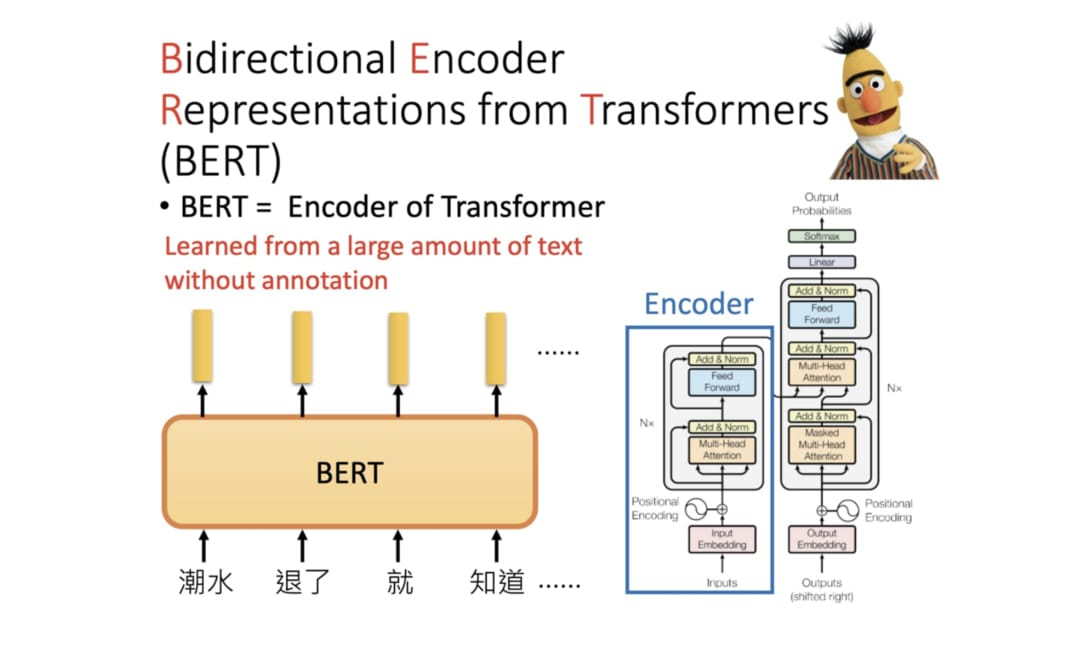Menu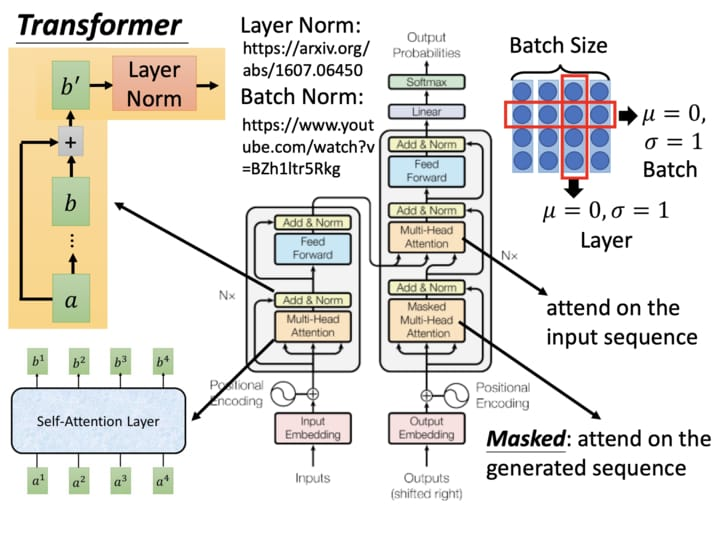─ 《創世記》第十一章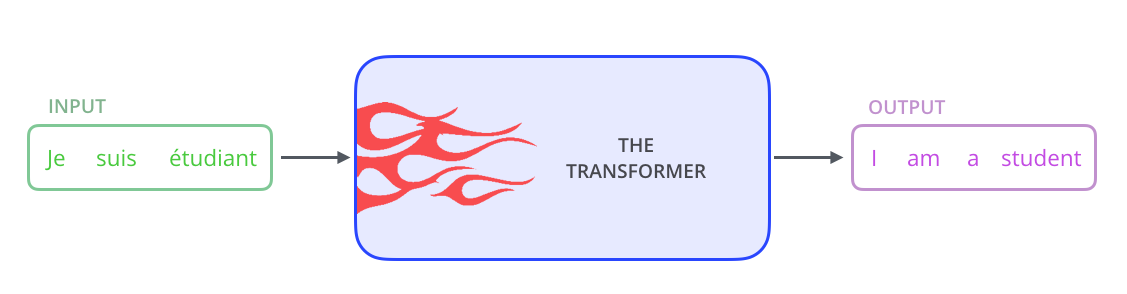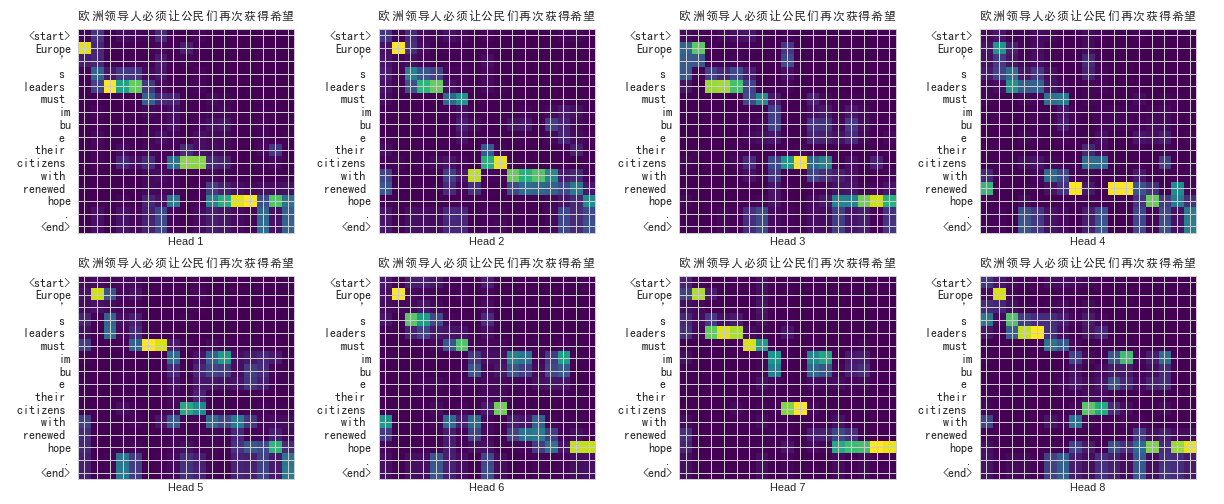Transformer 在將英文句子翻譯成中文時會「關注」需要注意的英文詞彙來生成對應的中文字詞

• 「必」、「須」時會關注「must」
• 「希」、「望」時會關注「hope」
• 「公」、「民」時會關注「citizens」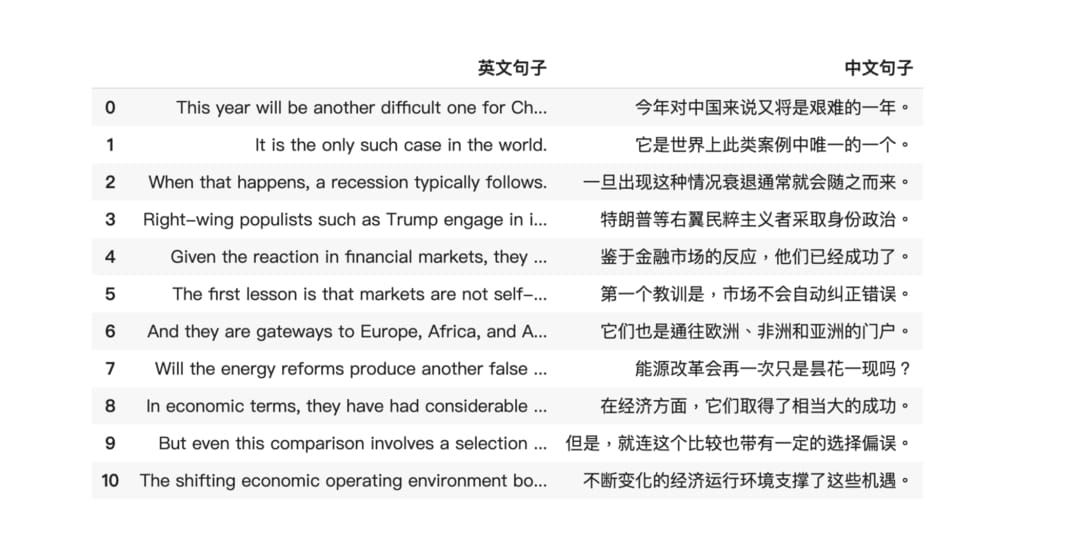## 一些你需先具備的基礎知識¶## 機器翻譯近代史¶

• 基於規則的機器翻譯 RBMT
• 基於範例的機器翻譯 EBMT
• 統計機器翻譯 SMT
• 近年的神經機器翻譯 NMT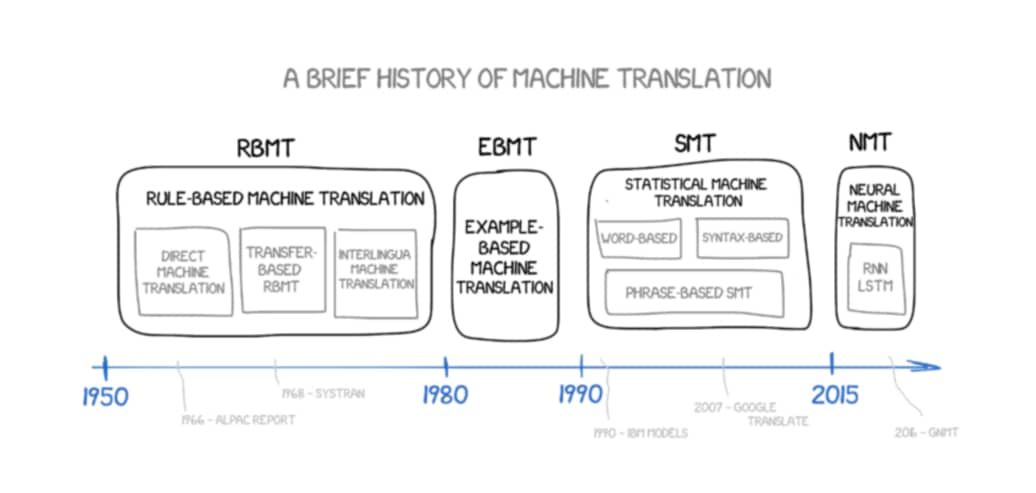### 統計機器翻譯：基於短語的翻譯¶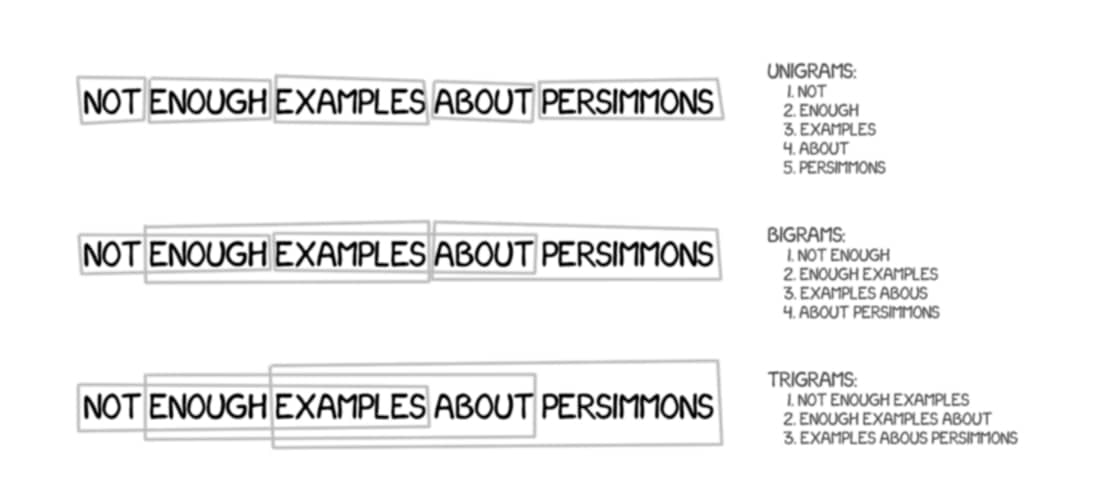PBMT 的翻譯結果相較於早年基於規則（Rule-Based）的手法已經進步很多，但仍然需要大量的平行語料、對齊語料來取得較好的結果。且因為是以短語為單位在做翻譯，這些短語拼湊出來的句子仍然不夠自然。

（如果你有當年 Google 翻譯結果的截圖的話歡迎提供）

### 神經機器翻譯：Encoder-Decoder 模型¶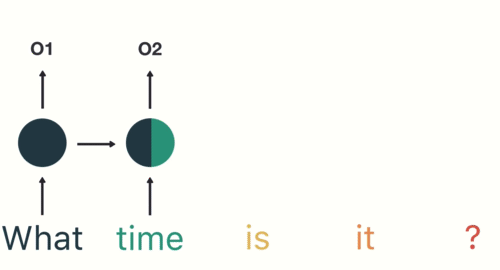RNN 很適合拿來處理具有時間順序的序列數據（下方的詞在丟入 RNN 前會被轉成詞向量） （圖片來源

Seq2Seq 模型裡頭 Encoder 跟 Decoder 是各自獨立的 RNN。Encoder 把輸入的句子做處理後所得到的隱狀態向量（圖中的 Hidden State#3）交給 Decoder 來生成目標語言。

• （Encoder）解譯來源文字的文意
• （Decoder）重新編譯該文意至目標語言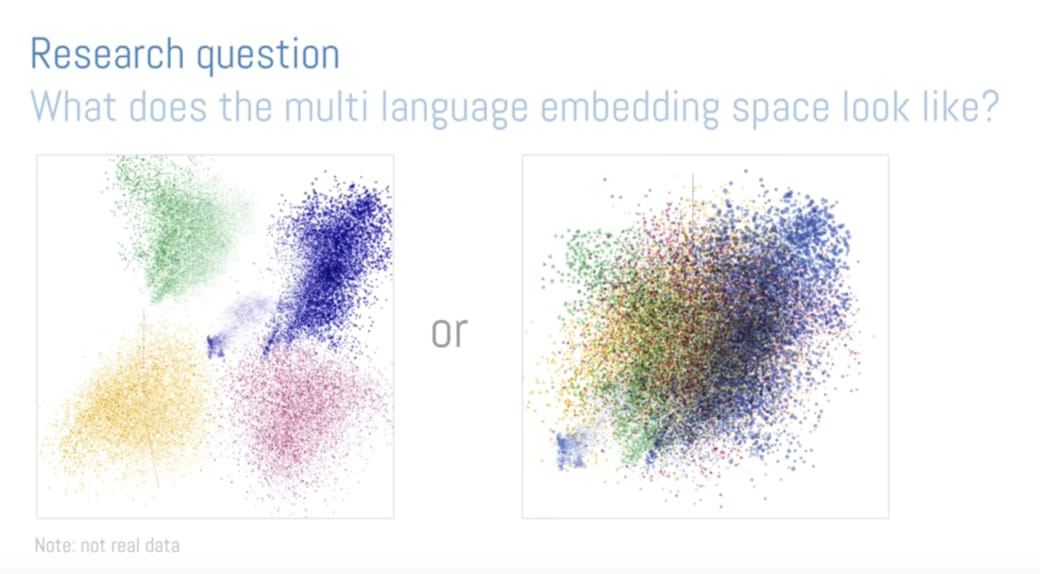Google 在 2016 年的研究結果發現，在此空間裡頭語言相異但擁有同語義的句子之間的距離 d1，要比同語言但不同語義的句子之間的距離 d2 要小得多（即 d1 << d2）。

英文：
From low-cost pharmacy brand moisturizers to high-priced cosmetics brand moisturizers, competition is fierce.

싸구려백화점화장품브랜드 moisturizers 에 저렴한약국브랜드 moisturizers 에서 , 경쟁이큰있습니다


### Encoder-Decoder 模型 + 注意力機制¶

• 呈現算好的注意程度而不是計算過程
• Encoder / 跟 Decoder 的實際架構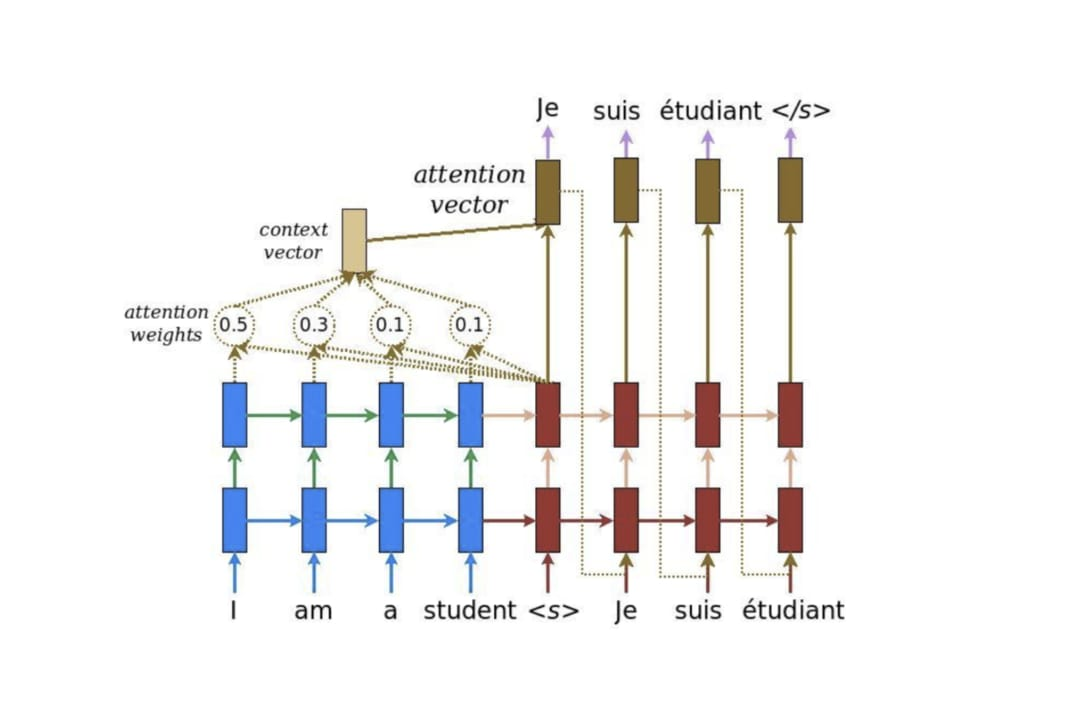1. 拿 Decoder 當下的紅色隱狀態向量 ht 跟 Encoder 所有藍色隱狀態向量 hs 做比較，利用 score 函式計算出 ht 對每個 hs 的注意程度
2. 以此注意程度為權重，加權平均所有 Encoder 隱狀態 hs 以取得上下文向量 context vector
3. 將此上下文向量與 Decoder 隱狀態結合成一個注意向量 attention vector 並作為該時間的輸出
4. 該注意向量會作為 Decoder 下個時間點的輸入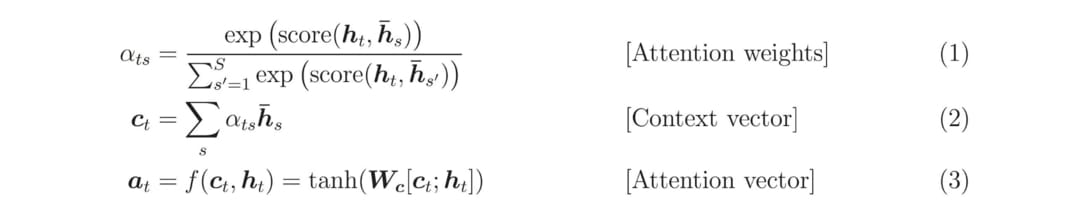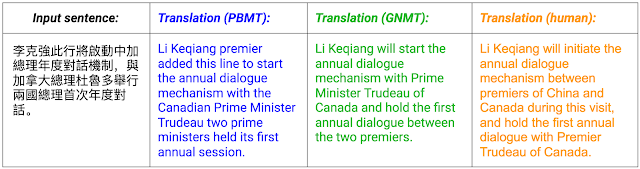NMT、PBMT 以及人類在中英翻譯時的結果比較 （圖片來源

### Transformer：Seq2Seq 模型 + 自注意力機制¶

Seq2Seq 模型搭配注意力機制感覺已經很猛了，難道還有什麼可以改善的嗎？

[a1, a2, a3, a4]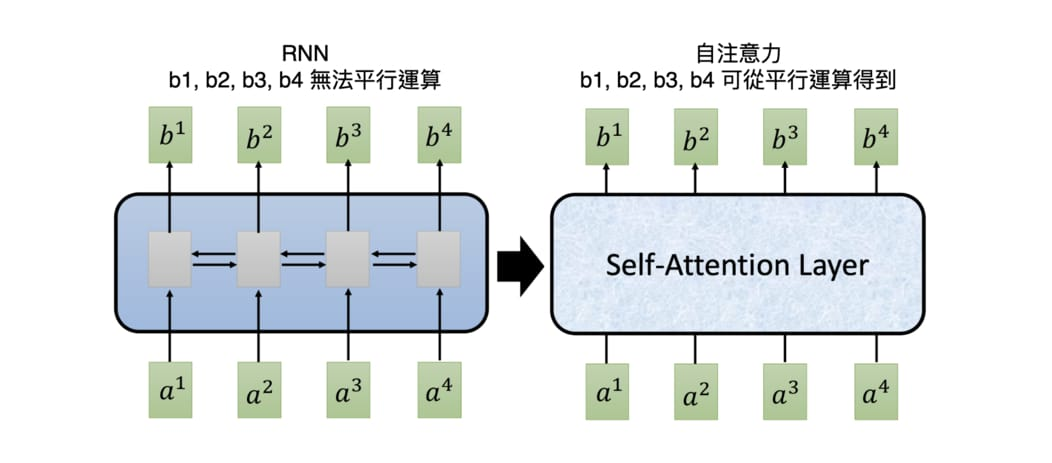Google 在 2017 年 6 月的一篇論文：Attention Is All You Need 裡參考了注意力機制，提出了注意力機制（Self-Attention mechanism）。這個機制不只跟 RNN 一樣可以處理序列數據，還可以平行運算。

[a1, a2, a3, a4]


repr. 為 representation 縮寫，在本文的機器翻譯情境裡頭，其意味著可以用來描述某個詞彙、句子意涵的多維實數張量。

• 注意力機制讓 Decoder 在生成輸出元素的 repr. 時關注 Encoder 的輸出序列，從中獲得上下文資訊
• 自注意力機制讓 Encoder 在生成輸入元素的 repr. 時關注自己序列中的其他元素，從中獲得上下文資訊
• 自注意力機制讓 Decoder 在生成輸出元素的 repr. 時關注自己序列中的其他元素，從中獲得上下文資訊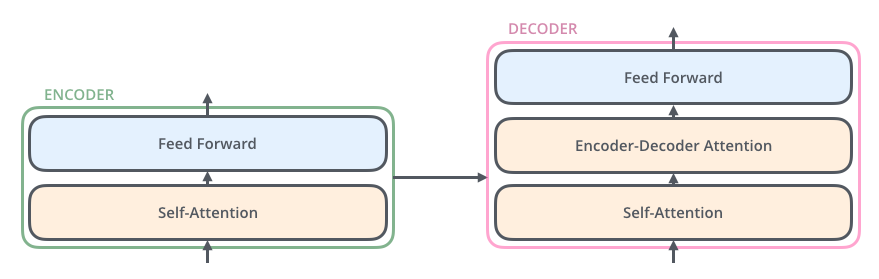1. Encoder 為輸入序列裡的每個詞彙產生初始的 repr. （即詞向量），以空圈表示
2. 利用自注意力機制將序列中所有詞彙的語義資訊各自匯總成每個詞彙的 repr.，以實圈表示
3. Encoder 重複 N 次自注意力機制，讓每個詞彙的 repr. 彼此持續修正以完整納入上下文語義
4. Decoder 在生成每個法文字時也運用了自注意力機制，關注自己之前已生成的元素，將其語義也納入之後生成的元素
5. 在自注意力機制後，Decoder 接著利用注意力機制關注 Encoder 的所有輸出並將其資訊納入當前生成元素的 repr.
6. Decoder 重複步驟 4, 5 以讓當前元素完整包含整體語義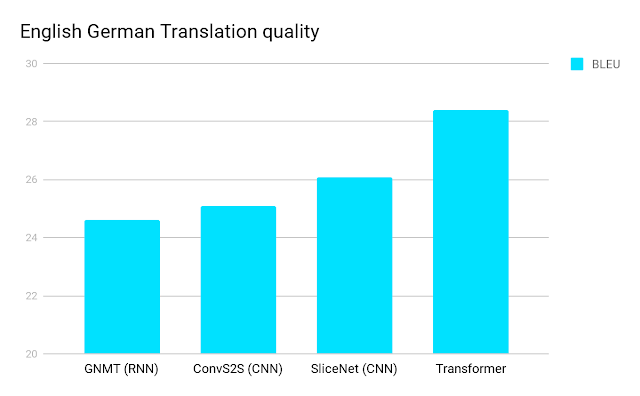Transformer 釋出時與其他模型在英德翻譯資料集上的比較 （圖片來源• 文本摘要（Text Summarization）
• 圖像描述（Image Captioning）
• 閱讀理解（Reading Comprehension）
• 語音辨識（Voice Recognition）
• 語言模型（Language Model）
• 聊天機器人（Chat Bot）
• 其他任何可以用 RNN 的潛在應用## 師傅引進門，修行在個人¶

• 想透過實作 Transformer 來徹底了解其內部運作原理的人
• 願意先花 1 小時了解 Transformer 的細節概念與理論的人TensorFlow 官方的 Transformer 教學## 11 個重要 Transformer 概念回顧¶

1. 自注意層（Self-Attention Layer）跟 RNN 一樣，輸入是一個序列，輸出一個序列。但是該層可以平行計算，且輸出序列中的每個向量都已經看了整個序列的資訊。

2. 自注意層將輸入序列 I 裡頭的每個位置的向量 i 透過 3 個線性轉換分別變成 3 個向量：qkv，並將每個位置的 q 拿去跟序列中其他位置的 k 做匹配，算出匹配程度後利用 softmax 層取得介於 0 到 1 之間的權重值，並以此權重跟每個位置的 v 作加權平均，最後取得該位置的輸出向量 o。全部位置的輸出向量可以同時平行計算，最後輸出序列 O

3. 計算匹配程度（注意）的方法不只一種，只要能吃進 2 個向量並吐出一個數值即可。但在 Transformer 論文原文是將 2 向量做 dot product 算匹配程度。

4. 我們可以透過大量矩陣運算以及 GPU 將概念 2 提到的注意力機制的計算全部平行化，加快訓練效率（也是本文實作的重點）。

5. 多頭注意力機制（Multi-head Attention）是將輸入序列中的每個位置的 qkv 切割成多個 qikivi 再分別各自進行注意力機制。各自處理完以後把所有結果串接並視情況降維。這樣的好處是能讓各個 head 各司其職，學會關注序列中不同位置在不同 representaton spaces 的資訊。

6. 自注意力機制這樣的計算的好處是「天涯若比鄰」：序列中每個位置都可以在 O(1) 的距離內關注任一其他位置的資訊，運算效率較雙向 RNN 優秀。

7. 自注意層可以取代 Seq2Seq 模型裡頭以 RNN 為基礎的 Encoder / Decoder，而實際上全部替換掉後就（大致上）是 Transformer。

8. 自注意力機制預設沒有「先後順序」的概念，而這也是為何其可以快速平行運算的原因。在進行如機器翻譯等序列生成任務時，我們需要額外加入位置編碼（Positioning Encoding）來加入順序資訊。而在 Transformer 原論文中此值為手設而非訓練出來的模型權重。

9. Transformer 是一個 Seq2Seq 模型，自然包含了 Encoder / Decoder，而 Encoder 及 Decoder 可以包含多層結構相同的 blocks，裡頭每層都會有 multi-head attention 以及 Feed Forward Network。

10. 在每個 Encoder / Decoder block 裡頭，我們還會使用殘差連結（Residual Connection）以及 Layer Normalization。這些能幫助模型穩定訓練。

11. Decoder 在關注 Encoder 輸出時會需要遮罩（mask）來避免看到未來資訊。我們後面會看到，事實上還會需要其他遮罩。

## 安裝函式庫並設置環境¶

import os
import time
import numpy as np
import matplotlib as mpl
import matplotlib.pyplot as plt
from pprint import pprint
from IPython.display import clear_output


!pip install tensorflow-gpu==2.0.0-beta0
clear_output()

import tensorflow as tf
import tensorflow_datasets as tfds
print(tf.__version__)

2.0.0-beta0


import logging
logging.basicConfig(level="error")

np.set_printoptions(suppress=True)


output_dir = "nmt"
en_vocab_file = os.path.join(output_dir, "en_vocab")
zh_vocab_file = os.path.join(output_dir, "zh_vocab")
checkpoint_path = os.path.join(output_dir, "checkpoints")
log_dir = os.path.join(output_dir, 'logs')
download_dir = "tensorflow-datasets/downloads"

if not os.path.exists(output_dir):
os.makedirs(output_dir)


## 建立輸入管道¶

### 下載並準備資料集¶

tmp_builder = tfds.builder("wmt19_translate/zh-en")
pprint(tmp_builder.subsets)

{NamedSplit('train'): ['newscommentary_v14',
'wikititles_v1',
'uncorpus_v1',
'casia2015',
'casict2011',
'casict2015',
'datum2015',
'datum2017',
'neu2017'],
NamedSplit('validation'): ['newstest2018']}


• 聯合國數據：uncorpus_v1
• 維基百科標題：wikititles_v1
• 新聞評論：newscommentary_v14

config = tfds.translate.wmt.WmtConfig(
version=tfds.core.Version('0.0.3', experiments={tfds.core.Experiment.S3: False}),
language_pair=("zh", "en"),
subsets={
tfds.Split.TRAIN: ["newscommentary_v14"]
}
)
builder = tfds.builder("wmt_translate", config=config)
builder.download_and_prepare(download_dir=download_dir)
clear_output()


• 下載包含原始數據的壓縮檔
• 解壓縮得到 CSV 檔案
• 逐行讀取該 CSV 裡頭所有中英句子
• 將不符合格式的 row 自動過濾
• Shuffle 數據
• 將原數據轉換成 TFRecord 數據以加速讀取

### 切割資料集¶

train_perc = 20
val_prec = 1
drop_prec = 100 - train_perc - val_prec

split = tfds.Split.TRAIN.subsplit([train_perc, val_prec, drop_prec])
split

(NamedSplit('train')(tfds.percent[0:20]),
NamedSplit('train')(tfds.percent[20:21]),
NamedSplit('train')(tfds.percent[21:100]))

• Split 1：20% 數據
• Split 2：1% 數據
• Split 3：79% 數據

examples = builder.as_dataset(split=split, as_supervised=True)
train_examples, val_examples, _ = examples
print(train_examples)
print(val_examples)

<_OptionsDataset shapes: ((), ()), types: (tf.string, tf.string)>
<_OptionsDataset shapes: ((), ()), types: (tf.string, tf.string)>


for en, zh in train_examples.take(3):
print(en)
print(zh)
print('-' * 10)

tf.Tensor(b'Making Do With More', shape=(), dtype=string)
tf.Tensor(b'\xe5\xa4\x9a\xe5\x8a\xb3\xe5\xba\x94\xe5\xa4\x9a\xe5\xbe\x97', shape=(), dtype=string)
----------
tf.Tensor(b'If the Putins, Erdo\xc4\x9fans, and Orb\xc3\xa1ns of the world want to continue to benefit economically from the open international system, they cannot simply make up their own rules.', shape=(), dtype=string)
tf.Tensor(b'\xe5\xa6\x82\xe6\x9e\x9c\xe6\x99\xae\xe4\xba\xac\xe3\x80\x81\xe5\x9f\x83\xe5\xb0\x94\xe5\xa4\x9a\xe5\xae\x89\xe5\x92\x8c\xe6\xac\xa7\xe5\xb0\x94\xe7\x8f\xad\xe5\xb8\x8c\xe6\x9c\x9b\xe7\xbb\xa7\xe7\xbb\xad\xe4\xba\xab\xe6\x9c\x89\xe5\xbc\x80\xe6\x94\xbe\xe5\x9b\xbd\xe9\x99\x85\xe4\xbd\x93\xe7\xb3\xbb\xe6\x8f\x90\xe4\xbe\x9b\xe7\x9a\x84\xe7\xbb\x8f\xe6\xb5\x8e\xe5\x88\xa9\xe7\x9b\x8a\xef\xbc\x8c\xe5\xb0\xb1\xe4\xb8\x8d\xe8\x83\xbd\xe7\xae\x80\xe5\x8d\x95\xe5\x9c\xb0\xe5\x88\xb6\xe5\xae\x9a\xe8\x87\xaa\xe5\xb7\xb1\xe7\x9a\x84\xe8\xa7\x84\xe5\x88\x99\xe3\x80\x82', shape=(), dtype=string)
----------
tf.Tensor(b'This ceiling can be raised only in a deep depression or other exceptional circumstances, allowing for counter-cyclical policy so long as it is agreed that the additional deficit is cyclical, rather than structural.', shape=(), dtype=string)
tf.Tensor(b'\xe5\x8f\xaa\xe6\x9c\x89\xe5\x9c\xa8\xe5\x8f\x91\xe7\x94\x9f\xe6\xb7\xb1\xe5\xba\xa6\xe8\x90\xa7\xe6\x9d\xa1\xe6\x88\x96\xe5\x85\xb6\xe4\xbb\x96\xe5\x8f\x8d\xe5\xb8\xb8\xe4\xba\x8b\xe4\xbb\xb6\xe6\x97\xb6\xef\xbc\x8c\xe8\xbf\x99\xe4\xb8\x80\xe4\xb8\x8a\xe9\x99\x90\xe6\x89\x8d\xe8\x83\xbd\xe5\x81\x9a\xe5\x87\xba\xe8\xb0\x83\xe6\x95\xb4\xef\xbc\x8c\xe4\xbb\xa5\xe4\xbe\xbf\xe8\xae\xa9\xe5\x8f\x8d\xe5\x91\xa8\xe6\x9c\x9f\xe6\x94\xbf\xe7\xad\x96\xe5\xae\x9e\xe6\x96\xbd\xe8\xb6\xb3\xe5\xa4\x9f\xe7\x9a\x84\xe9\x95\xbf\xe5\xba\xa6\xef\xbc\x8c\xe4\xbd\xbf\xe4\xba\xba\xe4\xbb\xac\xe4\xb8\x80\xe8\x87\xb4\xe8\xae\xa4\xe4\xb8\xba\xe5\xa2\x9e\xe5\x8a\xa0\xe7\x9a\x84\xe8\xb5\xa4\xe5\xad\x97\xe6\x98\xaf\xe5\x91\xa8\xe6\x9c\x9f\xe6\x80\xa7\xe7\x9a\x84\xef\xbc\x8c\xe8\x80\x8c\xe4\xb8\x8d\xe6\x98\xaf\xe7\xbb\x93\xe6\x9e\x84\xe6\x80\xa7\xe7\x9a\x84\xe3\x80\x82', shape=(), dtype=string)
----------


sample_examples = []
num_samples = 10

for en_t, zh_t in train_examples.take(num_samples):
en = en_t.numpy().decode("utf-8")
zh = zh_t.numpy().decode("utf-8")

print(en)
print(zh)
print('-' * 10)

# 之後用來簡單評估模型的訓練情況
sample_examples.append((en, zh))

Making Do With More

----------
If the Putins, Erdoğans, and Orbáns of the world want to continue to benefit economically from the open international system, they cannot simply make up their own rules.

----------
This ceiling can be raised only in a deep depression or other exceptional circumstances, allowing for counter-cyclical policy so long as it is agreed that the additional deficit is cyclical, rather than structural.

----------
Fascist and communist regimes of the past, which followed a similar instrumentalist approach to democracy, come to mind here.

----------
This phase culminated with the collapse of communism in 1989, but the chance to overcome the Continent’s historical divisions now required a redefinition of the European project.

----------
The eurozone’s collapse (and, for all practical purposes, that of the EU itself) forces a major realignment of European politics.

----------
With energy and enthusiasm, Burden turned that operation into a thriving health (not health-care) agency that covers three cities and about 300,000 people on the western edge of Los Angeles.

----------
The result could be a world of fragmented blocs – an outcome that would undermine not only global prosperity, but also cooperation on shared challenges.

----------
Among the questions being asked by NGOs, the UN, and national donors is how to prevent the recurrence of past mistakes.

----------
Managing the rise of NCDs will require long-term thinking, and government leaders will have to make investments that might pay off only after they are no longer in office.

----------


### 建立中文與英文字典¶

%%time
try:
subword_encoder_en = tfds.features.text.SubwordTextEncoder.load_from_file(en_vocab_file)
print(f"載入已建立的字典： {en_vocab_file}")
except:
print("沒有已建立的字典，從頭建立。")
subword_encoder_en = tfds.features.text.SubwordTextEncoder.build_from_corpus(
(en.numpy() for en, _ in train_examples),
target_vocab_size=2**13) # 有需要可以調整字典大小

# 將字典檔案存下以方便下次 warmstart
subword_encoder_en.save_to_file(en_vocab_file)

print(f"字典大小：{subword_encoder_en.vocab_size}")
print(f"前 10 個 subwords：{subword_encoder_en.subwords[:10]}")
print()

載入已建立的字典： /content/gdrive/My Drive/nmt/en_vocab

CPU times: user 41 ms, sys: 7.43 ms, total: 48.4 ms
Wall time: 391 ms


subword_encoder_en 則是利用 GNMT 當初推出的 wordpieces 來進行斷詞，而簡單來說其產生的子詞（subword）介於這兩者之間：

• 用英文字母分隔的斷詞（character-delimited）
• 用空白分隔的斷詞（word-delimited）

sample_string = 'Taiwan is beautiful.'
indices = subword_encoder_en.encode(sample_string)
indices

[2700, 7911, 10, 2942, 7457, 1163, 7925]

print("{0:10}{1:6}".format("Index", "Subword"))
print("-" * 15)
for idx in indices:
subword = subword_encoder_en.decode([idx])
print('{0:5}{1:6}'.format(idx, ' ' * 5 + subword))

Index     Subword
---------------
2700     Taiwan
7911
10     is
2942     bea
7457     uti
1163     ful
7925     .


sample_string = 'Taiwan is beautiful.'
indices = subword_encoder_en.encode(sample_string)
decoded_string = subword_encoder_en.decode(indices)
assert decoded_string == sample_string
pprint((sample_string, decoded_string))

('Taiwan is beautiful.', 'Taiwan is beautiful.')


%%time
try:
subword_encoder_zh = tfds.features.text.SubwordTextEncoder.load_from_file(zh_vocab_file)
print(f"載入已建立的字典： {zh_vocab_file}")
except:
print("沒有已建立的字典，從頭建立。")
subword_encoder_zh = tfds.features.text.SubwordTextEncoder.build_from_corpus(
(zh.numpy() for _, zh in train_examples),
target_vocab_size=2**13, # 有需要可以調整字典大小
max_subword_length=1) # 每一個中文字就是字典裡的一個單位

# 將字典檔案存下以方便下次 warmstart
subword_encoder_zh.save_to_file(zh_vocab_file)

print(f"字典大小：{subword_encoder_zh.vocab_size}")
print(f"前 10 個 subwords：{subword_encoder_zh.subwords[:10]}")
print()

載入已建立的字典： /content/gdrive/My Drive/nmt/zh_vocab

CPU times: user 27.6 ms, sys: 121 µs, total: 27.7 ms
Wall time: 337 ms


sample_string = sample_examples
indices = subword_encoder_zh.encode(sample_string)
print(sample_string)
print(indices)

多劳应多得
[48, 557, 116, 48, 81]


en = "The eurozone’s collapse forces a major realignment of European politics."
zh = "欧元区的瓦解强迫欧洲政治进行一次重大改组。"

# 將文字轉成為 subword indices
en_indices = subword_encoder_en.encode(en)
zh_indices = subword_encoder_zh.encode(zh)

print("[英中原文]（轉換前）")
print(en)
print(zh)
print()
print('-' * 20)
print()
print("[英中序列]（轉換後）")
print(en_indices)
print(zh_indices)

[英中原文]（轉換前）
The eurozone’s collapse forces a major realignment of European politics.

--------------------

[英中序列]（轉換後）
[17, 965, 11, 6, 1707, 676, 8, 211, 2712, 6683, 249, 3, 85, 1447, 7925]
[45, 206, 171, 1, 847, 197, 236, 604, 45, 90, 17, 130, 102, 36, 7, 284, 80, 18, 212, 265, 3]


### 前處理數據¶

• 開始 token、Begin of Sentence、BOS、<start>
• 結束 token、End of Sentence、EOS、<end>

def encode(en_t, zh_t):
# 因為字典的索引從 0 開始，
# 我們可以使用 subword_encoder_en.vocab_size 這個值作為 BOS 的索引值
# 用 subword_encoder_en.vocab_size + 1 作為 EOS 的索引值
en_indices = [subword_encoder_en.vocab_size] + subword_encoder_en.encode(
en_t.numpy()) + [subword_encoder_en.vocab_size + 1]
# 同理，不過是使用中文字典的最後一個索引 + 1
zh_indices = [subword_encoder_zh.vocab_size] + subword_encoder_zh.encode(
zh_t.numpy()) + [subword_encoder_zh.vocab_size + 1]

return en_indices, zh_indices


en_t, zh_t = next(iter(train_examples))
en_indices, zh_indices = encode(en_t, zh_t)
print('英文 BOS 的 index：', subword_encoder_en.vocab_size)
print('英文 EOS 的 index：', subword_encoder_en.vocab_size + 1)
print('中文 BOS 的 index：', subword_encoder_zh.vocab_size)
print('中文 EOS 的 index：', subword_encoder_zh.vocab_size + 1)

print('\n輸入為 2 個 Tensors：')
pprint((en_t, zh_t))
print('-' * 15)
print('輸出為 2 個索引序列：')
pprint((en_indices, zh_indices))

英文 BOS 的 index： 8135

(<tf.Tensor: id=306, shape=(), dtype=string, numpy=b'Making Do With More'>,
<tf.Tensor: id=307, shape=(), dtype=string, numpy=b'\xe5\xa4\x9a\xe5\x8a\xb3\xe5\xba\x94\xe5\xa4\x9a\xe5\xbe\x97'>)
---------------

([8135, 4682, 19, 717, 7911, 298, 2701, 7980, 8136],
[4201, 48, 557, 116, 48, 81, 4202])


train_dataset = train_examples.map(encode)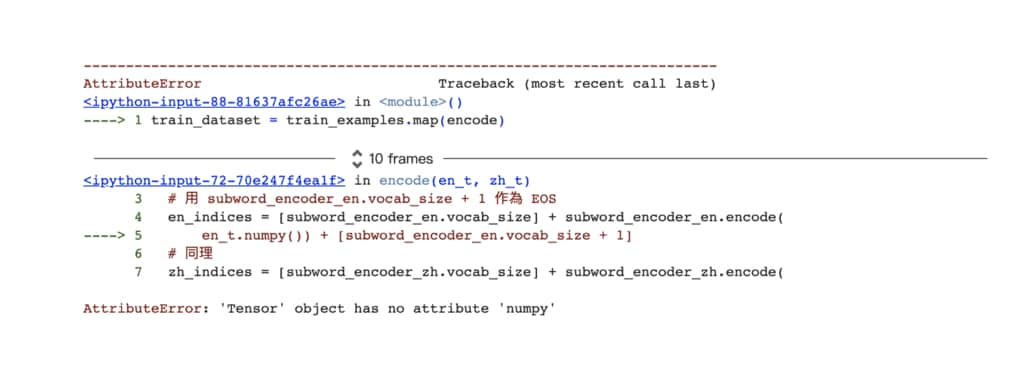def tf_encode(en_t, zh_t):
# 在 tf_encode 函式裡頭的 en_t 與 zh_t 都不是 Eager Tensors
# 要到 tf.py_funtion 裡頭才是
# 另外因為索引都是整數，所以使用 tf.int64
return tf.py_function(encode, [en_t, zh_t], [tf.int64, tf.int64])

# tmp_dataset 為說明用資料集，說明完所有重要的 func，
# 我們會從頭建立一個正式的 train_dataset
tmp_dataset = train_examples.map(tf_encode)
en_indices, zh_indices = next(iter(tmp_dataset))
print(en_indices)
print(zh_indices)

W0616 23:46:10.571188 140648854296320 backprop.py:842] The dtype of the watched tensor must be floating (e.g. tf.float32), got tf.string
W0616 23:46:10.573221 140648854296320 backprop.py:842] The dtype of the watched tensor must be floating (e.g. tf.float32), got tf.string

tf.Tensor([8135 4682   19  717 7911  298 2701 7980 8136], shape=(9,), dtype=int64)
tf.Tensor([4201   48  557  116   48   81 4202], shape=(7,), dtype=int64)


MAX_LENGTH = 40

def filter_max_length(en, zh, max_length=MAX_LENGTH):
# en, zh 分別代表英文與中文的索引序列
return tf.logical_and(tf.size(en) <= max_length,
tf.size(zh) <= max_length)

# tf.data.Dataset.filter(func) 只會回傳 func 為真的例子
tmp_dataset = tmp_dataset.filter(filter_max_length)


# 因為我們數據量小可以這樣 count
num_examples = 0
for en_indices, zh_indices in train_dataset:
cond1 = len(en_indices) <= MAX_LENGTH
cond2 = len(zh_indices) <= MAX_LENGTH
assert cond1 and cond2
num_examples += 1

print(f"所有英文與中文序列長度都不超過 {MAX_LENGTH} 個 tokens")
print(f"訓練資料集裡總共有 {num_examples} 筆數據")

訓練資料集裡總共有 29914 筆數據


BATCH_SIZE = 64
# 將 batch 裡的所有序列都 pad 到同樣長度
tmp_dataset = tmp_dataset.padded_batch(BATCH_SIZE, padded_shapes=([-1], [-1]))
en_batch, zh_batch = next(iter(tmp_dataset))
print("英文索引序列的 batch")
print(en_batch)
print('-' * 20)
print("中文索引序列的 batch")
print(zh_batch)

W0616 23:46:10.753194 140648845903616 backprop.py:842] The dtype of the watched tensor must be floating (e.g. tf.float32), got tf.string
W0616 23:46:10.760091 140648845903616 backprop.py:842] The dtype of the watched tensor must be floating (e.g. tf.float32), got tf.string
W0616 23:46:10.768630 140648845903616 backprop.py:842] The dtype of the watched tensor must be floating (e.g. tf.float32), got tf.string

英文索引序列的 batch
tf.Tensor(
[[8135 4682   19 ...    0    0    0]
[8135   17  965 ... 8136    0    0]
[8135 6602    2 ...    0    0    0]
...
[8135 1097  270 ...    0    0    0]
[8135 1713   70 ...    0    0    0]
[8135 2731 4553 ...    0    0    0]], shape=(64, 34), dtype=int64)
--------------------

tf.Tensor(
[[4201   48  557 ...    0    0    0]
[4201   45  206 ...    0    0    0]
[4201   58    5 ...  683    3 4202]
...
[4201   29  120 ...    0    0    0]
[4201  297  161 ...    0    0    0]
[4201  279  149 ... 4202    0    0]], shape=(64, 40), dtype=int64)


padded_batch 函式能幫我們將每個 batch 裡頭的序列都補 0 到跟當下 batch 裡頭最長的序列一樣長。

MAX_LENGTH = 40
BATCH_SIZE = 128
BUFFER_SIZE = 15000

# 訓練集
train_dataset = (train_examples  # 輸出：(英文句子, 中文句子)
.map(tf_encode) # 輸出：(英文索引序列, 中文索引序列)
.filter(filter_max_length) # 同上，且序列長度都不超過 40
.cache() # 加快讀取數據
.shuffle(BUFFER_SIZE) # 將例子洗牌確保隨機性
.padded_batch(BATCH_SIZE, # 將 batch 裡的序列都 pad 到一樣長度
padded_shapes=([-1], [-1]))
.prefetch(tf.data.experimental.AUTOTUNE)) # 加速
# 驗證集
val_dataset = (val_examples
.map(tf_encode)
.filter(filter_max_length)
.padded_batch(BATCH_SIZE,
padded_shapes=([-1], [-1])))


en_batch, zh_batch = next(iter(train_dataset))
print("英文索引序列的 batch")
print(en_batch)
print('-' * 20)
print("中文索引序列的 batch")
print(zh_batch)

英文索引序列的 batch
tf.Tensor(
[[8135  222    1 ...    0    0    0]
[8135 3812  162 ...    0    0    0]
[8135 6267  838 ...    0    0    0]
...
[8135   17 1042 ...    0    0    0]
[8135 7877 1165 ...    0    0    0]
[8135 6414 7911 ...    0    0    0]], shape=(128, 40), dtype=int64)
--------------------

tf.Tensor(
[[4201  109   54 ...    3 4202    0]
[4201   30    4 ...    0    0    0]
[4201  402    4 ...    0    0    0]
...
[4201  626  515 ...    0    0    0]
[4201   49  249 ...    0    0    0]
[4201  905  209 ...    0    0    0]], shape=(128, 40), dtype=int64)


• 一次回傳大小為 128 的 2 個 batch，分別包含 128 個英文、中文的索引序列
• 序列開頭皆為 BOS，英文的 BOS 索引是 8135；中文的 BOS 索引則為 4201
• 兩語言 batch 裡的序列都被「拉長」到我們先前定義的最長序列長度：40

## 理解 Transformer 之旅：跟著多維向量去冒險¶Welcome to matrix, 準備進入多維空間

demo_examples = [
("It is important.", "这很重要。"),
("The numbers speak for themselves.", "数字证明了一切。"),
]
pprint(demo_examples)

[('It is important.', '这很重要。'),
('The numbers speak for themselves.', '数字证明了一切。')]


batch_size = 2
demo_examples = tf.data.Dataset.from_tensor_slices((
[en for en, _ in demo_examples], [zh for _, zh in demo_examples]
))

# 將兩個句子透過之前定義的字典轉換成子詞的序列（sequence of subwords）
# 並添加 padding token: <pad> 來確保 batch 裡的句子有一樣長度
demo_dataset = demo_examples.map(tf_encode)\
.padded_batch(batch_size, padded_shapes=([-1], [-1]))

# 取出這個 demo dataset 裡唯一一個 batch
inp, tar = next(iter(demo_dataset))
print('inp:', inp)
print('' * 10)
print('tar:', tar)

inp: tf.Tensor(
[[8135  105   10 1304 7925 8136    0    0]
[8135   17 3905 6013   12 2572 7925 8136]], shape=(2, 8), dtype=int64)

tar: tf.Tensor(
[[4201   10  241   80   27    3 4202    0    0    0]
[4201  162  467  421  189   14    7  553    3 4202]], shape=(2, 10), dtype=int64)


2 維矩陣還很容易想像，但我擔心等到你進入 3 維空間後就會想放棄人生了。所以還是先讓我們用人類比較容易理解的方式來呈現這些數據。

### 視覺化原始句子¶

• 不管張量（Tensor）變幾維，其第一個維度 shape 永遠代表 batch_size，也就代表著句子的數目
• 不同句子我們用不同顏色表示，方便你之後對照這些句子在轉換前後的差異
• x 軸（橫軸）代表張量的最後一個維度 shape[-1]，以上例來說分別為 810
• x, y 軸上的標籤分別代表倒數兩個維度 shape[-2]shape[-1] 其所代表的物理含義，如圖中的句子子詞
• 圖中張量的 name 會對應到程式碼裡頭定義的變數名稱，方便你對照並理解實作邏輯。我也會秀出張量的 shape 幫助你想像該向量在多維空間的長相。一個簡單的例子是：(batch_size, tar_seq_len)

### 視覺化 3 維詞嵌入張量¶

# + 2 是因為我們額外加了 <start> 以及 <end> tokens
vocab_size_en = subword_encoder_en.vocab_size + 2
vocab_size_zh = subword_encoder_zh.vocab_size + 2

# 為了方便 demo, 將詞彙轉換到一個 4 維的詞嵌入空間
d_model = 4
embedding_layer_en = tf.keras.layers.Embedding(vocab_size_en, d_model)
embedding_layer_zh = tf.keras.layers.Embedding(vocab_size_zh, d_model)

emb_inp = embedding_layer_en(inp)
emb_tar = embedding_layer_zh(tar)
emb_inp, emb_tar

emb_inp: tf.Tensor(
[[[ 0.00695511 -0.03370368 -0.03656032 -0.03336458]
[-0.02707888 -0.03917687 -0.01213828  0.00909697]
[ 0.0355427   0.04111305  0.00751223 -0.01974255]
[ 0.02443342 -0.03273199  0.01267544  0.03127003]
[-0.04879753 -0.00119017 -0.00157104  0.01117355]
[-0.02148524 -0.03413673  0.00708324  0.0121879 ]
[-0.00680635  0.02136201 -0.02036932 -0.04211974]
[-0.00680635  0.02136201 -0.02036932 -0.04211974]]

[[ 0.00695511 -0.03370368 -0.03656032 -0.03336458]
[-0.0325227  -0.03433502 -0.01849879  0.01439226]
[ 0.00144588 -0.00377025 -0.00798036 -0.04099905]
[ 0.04524285  0.02524642 -0.00924555 -0.01368124]
[-0.0159062   0.01108797 -0.0177028  -0.0435766 ]
[ 0.00240784 -0.04652226  0.01821991 -0.04349295]
[-0.04879753 -0.00119017 -0.00157104  0.01117355]
[-0.02148524 -0.03413673  0.00708324  0.0121879 ]]], shape=(2, 8, 4), dtype=float32)
--------------------
emb_tar: tf.Tensor(
[[[-0.0441955  -0.01026772  0.03740635  0.02017349]
[ 0.02129837 -0.00746276  0.03881821 -0.01586295]
[-0.01179456  0.02825376  0.00738146  0.02963744]
[ 0.01171205  0.04350302 -0.01190796  0.02526634]
[ 0.03814722 -0.03364048 -0.03744673  0.04369817]
[ 0.0280853   0.01269842  0.04268574 -0.04069148]
[ 0.04029209 -0.00619308 -0.04934603  0.02242902]
[-0.00285894  0.02392108 -0.03126474  0.01345349]
[-0.00285894  0.02392108 -0.03126474  0.01345349]
[-0.00285894  0.02392108 -0.03126474  0.01345349]]

[[-0.0441955  -0.01026772  0.03740635  0.02017349]
[-0.00359621 -0.01380367 -0.02875998 -0.03855735]
[ 0.04516688 -0.04480755 -0.03278694 -0.0093614 ]
[ 0.04131394 -0.04065727 -0.04330624 -0.03341667]
[ 0.03572228 -0.04500845  0.0470326   0.03095007]
[-0.03566641 -0.03730996 -0.00597564 -0.03933349]
[ 0.01850356  0.03993076  0.02729526 -0.04848848]
[-0.02294568 -0.02494572 -0.0136737  -0.04278342]
[ 0.0280853   0.01269842  0.04268574 -0.04069148]
[ 0.04029209 -0.00619308 -0.04934603  0.02242902]]], shape=(2, 10, 4), dtype=float32)


print("tar:", tar[-3:])
print("-" * 20)
print("emb_tar:", emb_tar[-3:])

tar: tf.Tensor([0 0 0], shape=(3,), dtype=int64)
--------------------
emb_tar: tf.Tensor(
[[-0.00285894  0.02392108 -0.03126474  0.01345349]
[-0.00285894  0.02392108 -0.03126474  0.01345349]
[-0.00285894  0.02392108 -0.03126474  0.01345349]], shape=(3, 4), dtype=float32)


### 遮罩：Transformer 的祕密配方¶

• padding mask
• look ahead mask

padding mask 是讓 Transformer 用來識別序列實際的內容到哪裡。此遮罩負責的就是將序列中被補 0 的地方（也就是 <pad>）的位置蓋住，讓 Transformer 可以避免「關注」到這些位置。

look ahead mask 人如其名，是用來確保 Decoder 在進行自注意力機制時每個子詞只會「往前看」：關注（包含）自己之前的字詞，不會不小心關注「未來」Decoder 產生的子詞。我們後面還會看到 look ahead mask 的詳細介紹，但不管是哪一種遮罩向量，那些值為 1 的位置就是遮罩存在的地方。

def create_padding_mask(seq):
# padding mask 的工作就是把索引序列中為 0 的位置設為 1
mask = tf.cast(tf.equal(seq, 0), tf.float32)
return mask[:, tf.newaxis, tf.newaxis, :] #　broadcasting

inp_mask = create_padding_mask(inp)
inp_mask

<tf.Tensor: id=193029, shape=(2, 1, 1, 8), dtype=float32, numpy=
array([[[[0., 0., 0., 0., 0., 0., 1., 1.]]],

[[[0., 0., 0., 0., 0., 0., 0., 0.]]]], dtype=float32)>

print("inp:", inp)
print("-" * 20)
print("tf.squeeze(inp_mask):", tf.squeeze(inp_mask))

inp: tf.Tensor(
[[8135  105   10 1304 7925 8136    0    0]
[8135   17 3905 6013   12 2572 7925 8136]], shape=(2, 8), dtype=int64)
--------------------
tf.squeeze(inp_mask): tf.Tensor(
[[0. 0. 0. 0. 0. 0. 1. 1.]
[0. 0. 0. 0. 0. 0. 0. 0.]], shape=(2, 8), dtype=float32)


### Scaled dot product attention：一種注意函式¶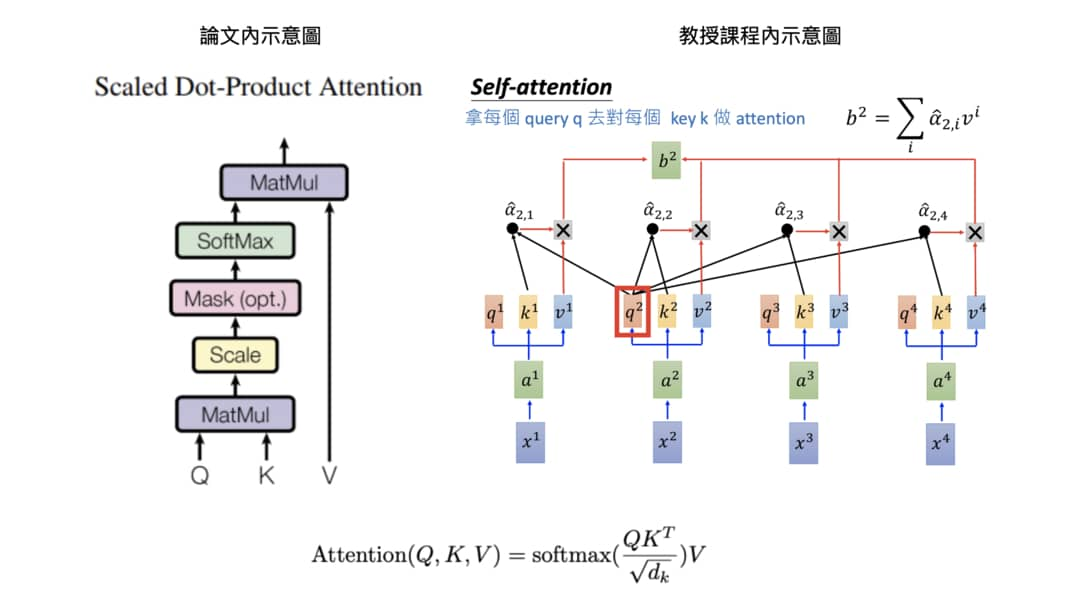Scaled dot product attention 跟以往 multiplicative attention 一樣是先將維度相同的 Q 跟 K 做點積：將對應維度的值兩兩相乘後相加得到單一數值，接著把這些數值除以一個 scaling factor sqrt(dk) ，然後再丟入 softmax 函式得到相加為 1 的注意權重（attention weights）。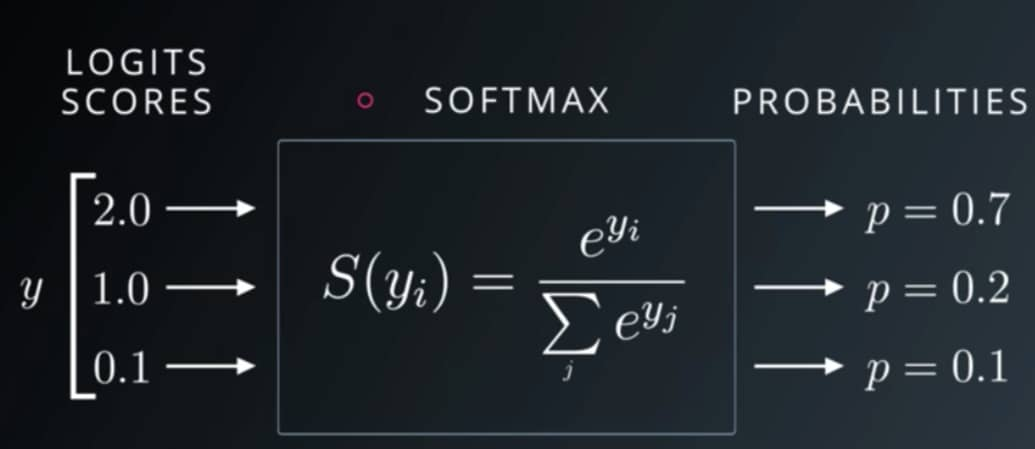Softmax 函式讓某個 Q 與多個 K 之間的匹配值和為 1

# 設定一個 seed 確保我們每次都拿到一樣的隨機結果
tf.random.set_seed(9527)

# 自注意力機制：查詢 q 跟鍵值 k 都是 emb_inp
q = emb_inp
k = emb_inp
# 簡單產生一個跟 emb_inp 同樣 shape 的 binary vector
v = tf.cast(tf.math.greater(tf.random.uniform(shape=emb_inp.shape), 0.5), tf.float32)
v

<tf.Tensor: id=193043, shape=(2, 8, 4), dtype=float32, numpy=
array([[[1., 0., 0., 0.],
[0., 1., 0., 1.],
[0., 0., 0., 1.],
[1., 0., 1., 0.],
[1., 0., 1., 0.],
[0., 1., 0., 1.],
[0., 0., 1., 0.],
[0., 1., 0., 1.]],

[[1., 0., 1., 1.],
[1., 0., 1., 0.],
[1., 0., 0., 0.],
[1., 0., 1., 0.],
[0., 1., 0., 1.],
[1., 1., 1., 1.],
[0., 0., 0., 0.],
[0., 0., 1., 0.]]], dtype=float32)>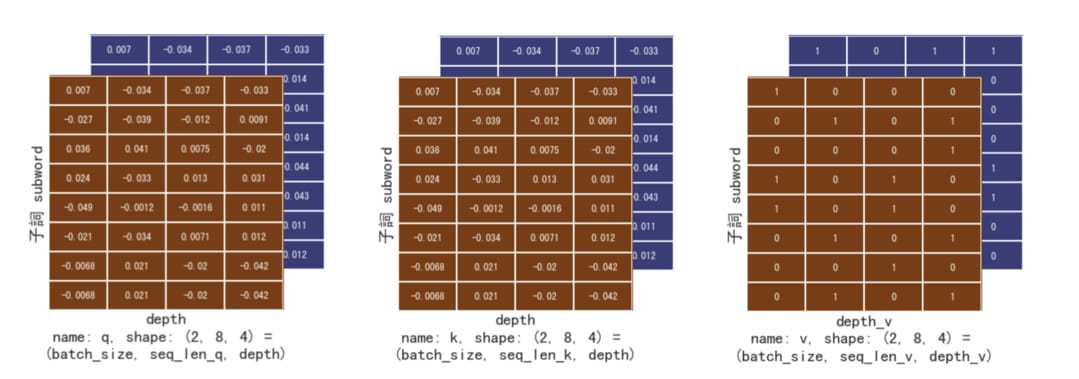def scaled_dot_product_attention(q, k, v, mask):
"""Calculate the attention weights.
q, k, v must have matching leading dimensions.
k, v must have matching penultimate dimension, i.e.: seq_len_k = seq_len_v.
The mask has different shapes depending on its type(padding or look ahead)
but it must be broadcastable for addition.

Args:
q: query shape == (..., seq_len_q, depth)
k: key shape == (..., seq_len_k, depth)
v: value shape == (..., seq_len_v, depth_v)
mask: Float tensor with shape broadcastable
to (..., seq_len_q, seq_len_k). Defaults to None.

Returns:
output, attention_weights
"""
# 將 q、 k 做點積再 scale
matmul_qk = tf.matmul(q, k, transpose_b=True)  # (..., seq_len_q, seq_len_k)

dk = tf.cast(tf.shape(k)[-1], tf.float32)  # 取得 seq_k 的序列長度
scaled_attention_logits = matmul_qk / tf.math.sqrt(dk)  # scale by sqrt(dk)

# 將遮罩「加」到被丟入 softmax 前的 logits
if mask is not None:
scaled_attention_logits += (mask * -1e9)

# 取 softmax 是為了得到總和為 1 的比例之後對 v 做加權平均
attention_weights = tf.nn.softmax(scaled_attention_logits, axis=-1)  # (..., seq_len_q, seq_len_k)

# 以注意權重對 v 做加權平均（weighted average）
output = tf.matmul(attention_weights, v)  # (..., seq_len_q, depth_v)

return output, attention_weights


1. qk 做點積得到 matmul_qk
2. matmul_qk 除以 scaling factor sqrt(dk)
3. 有遮罩的話在丟入 softmax 套用
4. 通過 softmax 取得加總為 1 的注意權重
5. 以該權重加權平均 v 作為輸出結果
6. 回傳輸出結果以及注意權重

mask = None
output, attention_weights = scaled_dot_product_attention(q, k, v, mask)
print("output:", output)
print("-" * 20)
print("attention_weights:", attention_weights)

output: tf.Tensor(
[[[0.37502408 0.37503672 0.37488326 0.49993956]
[0.37513658 0.37514552 0.37500778 0.49994028]
[0.37483314 0.37482613 0.3749625  0.50006175]
[0.37516367 0.37501514 0.3750258  0.49997073]
[0.37503195 0.3751256  0.3750621  0.49998796]
[0.37512696 0.37512186 0.37502852 0.49996266]
[0.3748441  0.3749599  0.37492597 0.50001484]
[0.3748441  0.3749599  0.37492597 0.50001484]]

[[0.62516296 0.2500847  0.6250717  0.37522966]
[0.62490153 0.24994145 0.62504375 0.37497035]
[0.62509674 0.2501282  0.6249581  0.37518966]
[0.62518024 0.25003165 0.6250133  0.37507355]
[0.6250232  0.25011832 0.62486345 0.37516582]
[0.6251376  0.25018096 0.625095   0.37525034]
[0.62478966 0.24995528 0.6248975  0.37490302]
[0.62492853 0.24997747 0.62507135 0.37497336]]], shape=(2, 8, 4), dtype=float32)
--------------------
attention_weights: tf.Tensor(
[[[0.12517719 0.12502946 0.12490283 0.12493535 0.12491155 0.12497091
0.12503636 0.12503636]
[0.12505189 0.12512855 0.12479477 0.1250193  0.12506542 0.12509388
0.12492308 0.12492308]
[0.12497574 0.12484524 0.1252356  0.12496044 0.12489695 0.1248758
0.12510511 0.12510511]
[0.12500346 0.12506503 0.1249556  0.12519364 0.12496658 0.12508455
0.12486558 0.12486558]
[0.12494988 0.12508136 0.12486238 0.12493681 0.12514524 0.12506418
0.12498005 0.12498005]
[0.12500885 0.12510943 0.12484082 0.12505434 0.12506378 0.12510203
0.12491038 0.12491038]
[0.1250592  0.12492351 0.12505497 0.12482036 0.12496454 0.12489527
0.12514108 0.12514108]
[0.1250592  0.12492351 0.12505497 0.12482036 0.12496454 0.12489527
0.12514108 0.12514108]]

[[0.12514497 0.1249882  0.12503006 0.12493392 0.1250188  0.12506588
0.1248794  0.12493874]
[0.1250289  0.12513264 0.12493595 0.12481083 0.12494826 0.12499319
0.12507208 0.12507817]
[0.12506142 0.12492662 0.12505917 0.12498691 0.12506557 0.12506266
0.12491715 0.12492047]
[0.12504192 0.12487808 0.1250636  0.12521076 0.12504579 0.12498584
0.12487733 0.12489669]
[0.12504749 0.12493626 0.12506288 0.12496644 0.12510824 0.12501009
0.12496544 0.12490314]
[0.12506938 0.12495602 0.1250348  0.12488137 0.12498492 0.12519602
0.12488527 0.12499221]
[0.12494776 0.12509981 0.1249542  0.12483776 0.12500516 0.12495013
0.12514311 0.12506206]
[0.12499588 0.12509465 0.12494626 0.12484587 0.1249316  0.12504588
0.12505081 0.12508905]]], shape=(2, 8, 8), dtype=float32)


scaled_dot_product_attention 函式輸出兩個張量：

• output 代表注意力機制的結果
• attention_weights 代表句子 q 裡頭每個子詞對句子 k 裡頭的每個子詞的注意權重

output 則是句子裡頭每個位置的子詞將 attention_weights 當作權重，從其他位置的子詞對應的資訊 v 裡頭抽取有用訊息後匯總出來的結果。你可以想像 ouput 裡頭的每個子詞都獲得了一個包含自己以及周遭子詞語義資訊的新 representation。而因為現在每個字詞的注意權重都相同，最後得到的每個 repr. 都長得一樣。

Scaled dot product attention 的實際運算過程

P.S. 一般注意函式只需輸出注意張量。而我們在這邊將注意權重 attention_weights 也輸出是為了方便之後觀察 Transformer 在訓練的時候將「注意力」放在哪裡。

### 直觀理解遮罩在注意函式中的效果¶

...

# 將 q、 k 做點積再 scale
scaled_attention_logits = matmul_qk / tf.math.sqrt(dk)

# 將遮罩「加」到被丟入 softmax 前的 logits
if mask is not None:
scaled_attention_logits += (mask * -1e9)

# 取 softmax 是為了得到總和為 1 的比例做加權平均
attention_weights = tf.nn.softmax(scaled_attention_logits, axis=-1)

...


...

if mask is not None:
scaled_attention_logits += (mask * -1e9) # 是 -1e9 不是 1e-9

attention_weights = tf.nn.softmax(scaled_attention_logits, axis=-1)

...


（動腦時間：為何遮罩要放在 softmax 之前而不能放之後？）

def create_padding_mask(seq):
# padding mask 的工作就是把索引序列中為 0 的位置設為 1
mask = tf.cast(tf.equal(seq, 0), tf.float32)
return mask[:, tf.newaxis, tf.newaxis, :] #　broadcasting

print("inp:", inp)
inp_mask = create_padding_mask(inp)
print("-" * 20)
print("inp_mask:", inp_mask)

inp: tf.Tensor(
[[8135  105   10 1304 7925 8136    0    0]
[8135   17 3905 6013   12 2572 7925 8136]], shape=(2, 8), dtype=int64)
--------------------
inp_mask: tf.Tensor(
[[[[0. 0. 0. 0. 0. 0. 1. 1.]]]

[[[0. 0. 0. 0. 0. 0. 0. 0.]]]], shape=(2, 1, 1, 8), dtype=float32)


# 這次讓我們將 padding mask 放入注意函式並觀察
# 注意權重的變化
mask = tf.squeeze(inp_mask, axis=1) # (batch_size, 1, seq_len_q)
_, attention_weights = scaled_dot_product_attention(q, k, v, mask)
print("attention_weights:", attention_weights)

attention_weights: tf.Tensor(
[[[0.16691911 0.1667221  0.16655324 0.16659662 0.1665649  0.16664404
0.         0.        ]
[0.16670164 0.16680385 0.1663589  0.16665822 0.16671969 0.16675764
0.         0.        ]
[0.16668104 0.16650699 0.16702762 0.16666064 0.16657597 0.16654775
0.         0.        ]
[0.16661155 0.16669361 0.16654776 0.16686502 0.16656238 0.16671962
0.         0.        ]
[0.16659099 0.16676629 0.16647433 0.16657357 0.16685146 0.16674338
0.         0.        ]
[0.16663864 0.16677272 0.16641466 0.16669929 0.16671185 0.16676286
0.         0.        ]
[0.16680835 0.16662736 0.1668027  0.16648975 0.16668208 0.1665897
0.         0.        ]
[0.16680835 0.16662736 0.1668027  0.16648975 0.16668208 0.1665897
0.         0.        ]]

[[0.12514497 0.1249882  0.12503006 0.12493392 0.1250188  0.12506588
0.1248794  0.12493874]
[0.1250289  0.12513264 0.12493595 0.12481083 0.12494826 0.12499319
0.12507208 0.12507817]
[0.12506142 0.12492662 0.12505917 0.12498691 0.12506557 0.12506266
0.12491715 0.12492047]
[0.12504192 0.12487808 0.1250636  0.12521076 0.12504579 0.12498584
0.12487733 0.12489669]
[0.12504749 0.12493626 0.12506288 0.12496644 0.12510824 0.12501009
0.12496544 0.12490314]
[0.12506938 0.12495602 0.1250348  0.12488137 0.12498492 0.12519602
0.12488527 0.12499221]
[0.12494776 0.12509981 0.1249542  0.12483776 0.12500516 0.12495013
0.12514311 0.12506206]
[0.12499588 0.12509465 0.12494626 0.12484587 0.1249316  0.12504588
0.12505081 0.12508905]]], shape=(2, 8, 8), dtype=float32)


# 事實上也不完全是上句話的翻譯，
# 因為我們在第一個維度還是把兩個句子都拿出來方便你比較
attention_weights[:, :, -2:]

<tf.Tensor: id=193086, shape=(2, 8, 2), dtype=float32, numpy=
array([[[0.        , 0.        ],
[0.        , 0.        ],
[0.        , 0.        ],
[0.        , 0.        ],
[0.        , 0.        ],
[0.        , 0.        ],
[0.        , 0.        ],
[0.        , 0.        ]],

[[0.1248794 , 0.12493874],
[0.12507208, 0.12507817],
[0.12491715, 0.12492047],
[0.12487733, 0.12489669],
[0.12496544, 0.12490314],
[0.12488527, 0.12499221],
[0.12514311, 0.12506206],
[0.12505081, 0.12508905]]], dtype=float32)>

# 建立一個 2 維矩陣，維度為 (size, size)，
# 其遮罩為一個右上角的三角形
def create_look_ahead_mask(size):
mask = 1 - tf.linalg.band_part(tf.ones((size, size)), -1, 0)
return mask  # (seq_len, seq_len)

seq_len = emb_tar.shape # 注意這次我們用中文的詞嵌入張量 emb_tar
look_ahead_mask = create_look_ahead_mask(seq_len)
print("emb_tar:", emb_tar)
print("-" * 20)
print("look_ahead_mask", look_ahead_mask)

emb_tar: tf.Tensor(
[[[-0.0441955  -0.01026772  0.03740635  0.02017349]
[ 0.02129837 -0.00746276  0.03881821 -0.01586295]
[-0.01179456  0.02825376  0.00738146  0.02963744]
[ 0.01171205  0.04350302 -0.01190796  0.02526634]
[ 0.03814722 -0.03364048 -0.03744673  0.04369817]
[ 0.0280853   0.01269842  0.04268574 -0.04069148]
[ 0.04029209 -0.00619308 -0.04934603  0.02242902]
[-0.00285894  0.02392108 -0.03126474  0.01345349]
[-0.00285894  0.02392108 -0.03126474  0.01345349]
[-0.00285894  0.02392108 -0.03126474  0.01345349]]

[[-0.0441955  -0.01026772  0.03740635  0.02017349]
[-0.00359621 -0.01380367 -0.02875998 -0.03855735]
[ 0.04516688 -0.04480755 -0.03278694 -0.0093614 ]
[ 0.04131394 -0.04065727 -0.04330624 -0.03341667]
[ 0.03572228 -0.04500845  0.0470326   0.03095007]
[-0.03566641 -0.03730996 -0.00597564 -0.03933349]
[ 0.01850356  0.03993076  0.02729526 -0.04848848]
[-0.02294568 -0.02494572 -0.0136737  -0.04278342]
[ 0.0280853   0.01269842  0.04268574 -0.04069148]
[ 0.04029209 -0.00619308 -0.04934603  0.02242902]]], shape=(2, 10, 4), dtype=float32)
--------------------
look_ahead_mask tf.Tensor(
[[0. 1. 1. 1. 1. 1. 1. 1. 1. 1.]
[0. 0. 1. 1. 1. 1. 1. 1. 1. 1.]
[0. 0. 0. 1. 1. 1. 1. 1. 1. 1.]
[0. 0. 0. 0. 1. 1. 1. 1. 1. 1.]
[0. 0. 0. 0. 0. 1. 1. 1. 1. 1.]
[0. 0. 0. 0. 0. 0. 1. 1. 1. 1.]
[0. 0. 0. 0. 0. 0. 0. 1. 1. 1.]
[0. 0. 0. 0. 0. 0. 0. 0. 1. 1.]
[0. 0. 0. 0. 0. 0. 0. 0. 0. 1.]
[0. 0. 0. 0. 0. 0. 0. 0. 0. 0.]], shape=(10, 10), dtype=float32)


# 讓我們用目標語言（中文）的 batch
# 來模擬 Decoder 處理的情況
temp_q = temp_k = emb_tar
temp_v = tf.cast(tf.math.greater(
tf.random.uniform(shape=emb_tar.shape), 0.5), tf.float32)

# 將 look_ahead_mask 放入注意函式
_, attention_weights = scaled_dot_product_attention(
temp_q, temp_k, temp_v, look_ahead_mask)

print("attention_weights:", attention_weights)

attention_weights: tf.Tensor(
[[[1.         0.         0.         0.         0.         0.
0.         0.         0.         0.        ]
[0.49974996 0.50025004 0.         0.         0.         0.
0.         0.         0.         0.        ]
[0.33338806 0.33309633 0.3335156  0.         0.         0.
0.         0.         0.         0.        ]
[0.24980238 0.2497976  0.25013384 0.25026616 0.         0.
0.         0.         0.         0.        ]
[0.19975185 0.19982941 0.19989952 0.199991   0.20052823 0.
0.         0.         0.         0.        ]
[0.16658378 0.16686733 0.16656147 0.16657883 0.1664059  0.16700274
0.         0.         0.         0.        ]
[0.14259693 0.1427213  0.14279391 0.1429158  0.14314583 0.14267854
0.14314772 0.         0.         0.        ]
[0.12491751 0.12487698 0.12503591 0.12508857 0.12503389 0.12487747
0.12507991 0.12508978 0.         0.        ]
[0.11102892 0.1109929  0.11113416 0.11118097 0.11113235 0.11099333
0.11117328 0.11118205 0.11118205 0.        ]
[0.09991965 0.09988723 0.10001437 0.10005648 0.10001273 0.09988762
0.10004956 0.10005745 0.10005745 0.10005745]]

[[1.         0.         0.         0.         0.         0.
0.         0.         0.         0.        ]
[0.4994912  0.5005088  0.         0.         0.         0.
0.         0.         0.         0.        ]
[0.33261845 0.33340293 0.3339786  0.         0.         0.
0.         0.         0.         0.        ]
[0.24919374 0.25002357 0.25033304 0.25044966 0.         0.
0.         0.         0.         0.        ]
[0.19997214 0.19964042 0.20002526 0.19986893 0.20049322 0.
0.         0.         0.         0.        ]
[0.16662474 0.16674054 0.16659829 0.16668092 0.16645522 0.16690029
0.         0.         0.         0.        ]
[0.14276491 0.14288287 0.14274995 0.14281946 0.1427529  0.14282054
0.14320944 0.         0.         0.        ]
[0.12491003 0.1250709  0.12497466 0.12504703 0.12481265 0.1251362
0.12493407 0.12511446 0.         0.        ]
[0.11102156 0.11105824 0.11103692 0.11106326 0.11112017 0.11104742
0.11128615 0.11106552 0.11130078 0.        ]
[0.09983386 0.10001399 0.10016464 0.10015456 0.09999382 0.09989963
0.0998925  0.09993652 0.099891   0.10021948]]], shape=(2, 10, 10), dtype=float32)


attention_weights[:, 0, :]

<tf.Tensor: id=193126, shape=(2, 10), dtype=float32, numpy=
array([[1., 0., 0., 0., 0., 0., 0., 0., 0., 0.],
[1., 0., 0., 0., 0., 0., 0., 0., 0., 0.]], dtype=float32)>

attention_weights[:, 1, :]

<tf.Tensor: id=193131, shape=(2, 10), dtype=float32, numpy=
array([[0.49974996, 0.50025004, 0.        , 0.        , 0.        ,
0.        , 0.        , 0.        , 0.        , 0.        ],
[0.4994912 , 0.5005088 , 0.        , 0.        , 0.        ,
0.        , 0.        , 0.        , 0.        , 0.        ]],
dtype=float32)>

look ahead mask 讓每個子詞都只關注序列中自己與之前的位置

• padding mask：遮住 <pad> token 不讓所有子詞關注
• look ahead mask：遮住 Decoder 未來生成的子詞不讓之前的子詞關注### Multi-head attention：你看你的，我看我的¶• Head 4 在生成「們」與「再」時特別關注「renewed」
• Head 5 在生成「必」與「須」時特別關注「must」
• Head 6 & 8 在生成「希」與「望」時特別關注「hope」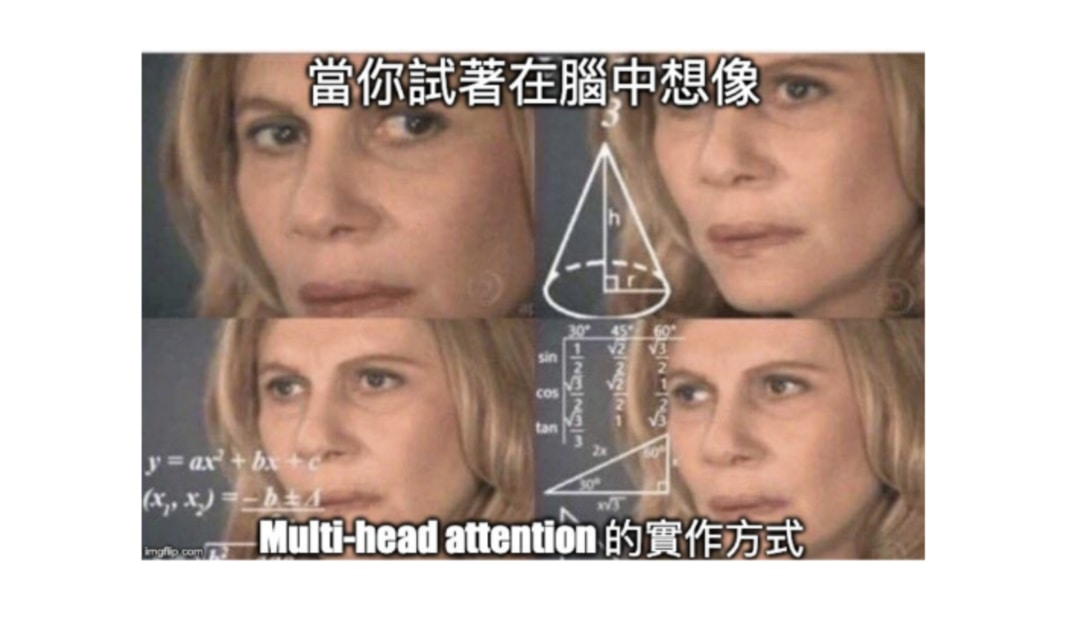num_heads * depth = d_model


def split_heads(x, d_model, num_heads):
# x.shape: (batch_size, seq_len, d_model)
batch_size = tf.shape(x)

# 我們要確保維度 d_model 可以被平分成 num_heads 個 depth 維度
assert d_model % num_heads == 0
depth = d_model // num_heads  # 這是分成多頭以後每個向量的維度

# 將最後一個 d_model 維度分成 num_heads 個 depth 維度。
# 最後一個維度變成兩個維度，張量 x 從 3 維到 4 維
# (batch_size, seq_len, num_heads, depth)
reshaped_x = tf.reshape(x, shape=(batch_size, -1, num_heads, depth))

# 將 head 的維度拉前使得最後兩個維度為子詞以及其對應的 depth 向量
# (batch_size, num_heads, seq_len, depth)
output = tf.transpose(reshaped_x, perm=[0, 2, 1, 3])

return output

# 我們的 emb_inp 裡頭的子詞本來就是 4 維的詞嵌入向量
d_model = 4
# 將 4 維詞嵌入向量分為 2 個 head 的 2 維矩陣
num_heads = 2
x = emb_inp

output = split_heads(x, d_model, num_heads)
print("x:", x)
print("output:", output)

x: tf.Tensor(
[[[ 0.00695511 -0.03370368 -0.03656032 -0.03336458]
[-0.02707888 -0.03917687 -0.01213828  0.00909697]
[ 0.0355427   0.04111305  0.00751223 -0.01974255]
[ 0.02443342 -0.03273199  0.01267544  0.03127003]
[-0.04879753 -0.00119017 -0.00157104  0.01117355]
[-0.02148524 -0.03413673  0.00708324  0.0121879 ]
[-0.00680635  0.02136201 -0.02036932 -0.04211974]
[-0.00680635  0.02136201 -0.02036932 -0.04211974]]

[[ 0.00695511 -0.03370368 -0.03656032 -0.03336458]
[-0.0325227  -0.03433502 -0.01849879  0.01439226]
[ 0.00144588 -0.00377025 -0.00798036 -0.04099905]
[ 0.04524285  0.02524642 -0.00924555 -0.01368124]
[-0.0159062   0.01108797 -0.0177028  -0.0435766 ]
[ 0.00240784 -0.04652226  0.01821991 -0.04349295]
[-0.04879753 -0.00119017 -0.00157104  0.01117355]
[-0.02148524 -0.03413673  0.00708324  0.0121879 ]]], shape=(2, 8, 4), dtype=float32)
output: tf.Tensor(
[[[[ 0.00695511 -0.03370368]
[-0.02707888 -0.03917687]
[ 0.0355427   0.04111305]
[ 0.02443342 -0.03273199]
[-0.04879753 -0.00119017]
[-0.02148524 -0.03413673]
[-0.00680635  0.02136201]
[-0.00680635  0.02136201]]

[[-0.03656032 -0.03336458]
[-0.01213828  0.00909697]
[ 0.00751223 -0.01974255]
[ 0.01267544  0.03127003]
[-0.00157104  0.01117355]
[ 0.00708324  0.0121879 ]
[-0.02036932 -0.04211974]
[-0.02036932 -0.04211974]]]

[[[ 0.00695511 -0.03370368]
[-0.0325227  -0.03433502]
[ 0.00144588 -0.00377025]
[ 0.04524285  0.02524642]
[-0.0159062   0.01108797]
[ 0.00240784 -0.04652226]
[-0.04879753 -0.00119017]
[-0.02148524 -0.03413673]]

[[-0.03656032 -0.03336458]
[-0.01849879  0.01439226]
[-0.00798036 -0.04099905]
[-0.00924555 -0.01368124]
[-0.0177028  -0.0435766 ]
[ 0.01821991 -0.04349295]
[-0.00157104  0.01117355]
[ 0.00708324  0.0121879 ]]]], shape=(2, 2, 8, 2), dtype=float32)


split_heads 函式將 3 維張量轉換為 multi-head 的 4 維張量過程

# 實作一個執行多頭注意力機制的 keras layer
# 在初始的時候指定輸出維度 d_model & num_heads，
# 在呼叫的時候輸入 v, k, q 以及 mask
# 輸出跟 scaled_dot_product_attention 函式一樣有兩個：
# output.shape            == (batch_size, seq_len_q, d_model)
# attention_weights.shape == (batch_size, num_heads, seq_len_q, seq_len_k)
class MultiHeadAttention(tf.keras.layers.Layer):
# 在初始的時候建立一些必要參數
def __init__(self, d_model, num_heads):
super(MultiHeadAttention, self).__init__()
self.num_heads = num_heads # 指定要將 d_model 拆成幾個 heads
self.d_model = d_model # 在 split_heads 之前的基底維度

assert d_model % self.num_heads == 0  # 前面看過，要確保可以平分

self.depth = d_model // self.num_heads  # 每個 head 裡子詞的新的 repr. 維度

self.wq = tf.keras.layers.Dense(d_model)  # 分別給 q, k, v 的 3 個線性轉換
self.wk = tf.keras.layers.Dense(d_model)  # 注意我們並沒有指定 activation func
self.wv = tf.keras.layers.Dense(d_model)

self.dense = tf.keras.layers.Dense(d_model)  # 多 heads 串接後通過的線性轉換

# 這跟我們前面看過的函式有 87% 相似
def split_heads(self, x, batch_size):
"""Split the last dimension into (num_heads, depth).
Transpose the result such that the shape is (batch_size, num_heads, seq_len, depth)
"""
x = tf.reshape(x, (batch_size, -1, self.num_heads, self.depth))
return tf.transpose(x, perm=[0, 2, 1, 3])

# multi-head attention 的實際執行流程，注意參數順序（這邊跟論文以及 TensorFlow 官方教學一致）
def call(self, v, k, q, mask):
batch_size = tf.shape(q)

# 將輸入的 q, k, v 都各自做一次線性轉換到 d_model 維空間
q = self.wq(q)  # (batch_size, seq_len, d_model)
k = self.wk(k)  # (batch_size, seq_len, d_model)
v = self.wv(v)  # (batch_size, seq_len, d_model)

# 前面看過的，將最後一個 d_model 維度分成 num_heads 個 depth 維度
q = self.split_heads(q, batch_size)  # (batch_size, num_heads, seq_len_q, depth)
k = self.split_heads(k, batch_size)  # (batch_size, num_heads, seq_len_k, depth)
v = self.split_heads(v, batch_size)  # (batch_size, num_heads, seq_len_v, depth)

# 利用 broadcasting 讓每個句子的每個 head 的 qi, ki, vi 都各自進行注意力機制
# 輸出會多一個 head 維度
scaled_attention, attention_weights = scaled_dot_product_attention(
q, k, v, mask)
# scaled_attention.shape == (batch_size, num_heads, seq_len_q, depth)
# attention_weights.shape == (batch_size, num_heads, seq_len_q, seq_len_k)

# 跟我們在 split_heads 函式做的事情剛好相反，先做 transpose 再做 reshape
# 將 num_heads 個 depth 維度串接回原來的 d_model 維度
scaled_attention = tf.transpose(scaled_attention, perm=[0, 2, 1, 3])
# (batch_size, seq_len_q, num_heads, depth)
concat_attention = tf.reshape(scaled_attention,
(batch_size, -1, self.d_model))
# (batch_size, seq_len_q, d_model)

# 通過最後一個線性轉換
output = self.dense(concat_attention)  # (batch_size, seq_len_q, d_model)

return output, attention_weights


split_heads 函式我們在前面就已經看過了，你應該還有印象。call 函式則定義了這個 multi-head attention layer 實際的計算流程，而這流程跟我在本節開頭講的可以說是有 87% 相似：

# emb_inp.shape == (batch_size, seq_len, d_model)
#               == (2, 8, 4)
assert d_model == emb_inp.shape[-1]  == 4
num_heads = 2

print(f"d_model: {d_model}")
print(f"num_heads: {num_heads}\n")

# 初始化一個 multi-head attention layer
mha = MultiHeadAttention(d_model, num_heads)

# 簡單將 v, k, q 都設置為 emb_inp
# 順便看看 padding mask 的作用。
# 別忘記，第一個英文序列的最後兩個 tokens 是 <pad>
v = k = q = emb_inp
padding_mask = create_padding_mask(inp)
print("q.shape:", q.shape)
print("k.shape:", k.shape)
print("v.shape:", v.shape)
print("padding_mask.shape:", padding_mask.shape)

output, attention_weights = mha(v, k, q, mask)
print("output.shape:", output.shape)
print("attention_weights.shape:", attention_weights.shape)

print("\noutput:", output)

d_model: 4
num_heads: 2

q.shape: (2, 8, 4)
k.shape: (2, 8, 4)
v.shape: (2, 8, 4)
padding_mask.shape: (2, 1, 1, 8)
output.shape: (2, 8, 4)
attention_weights.shape: (2, 2, 8, 8)

output: tf.Tensor(
[[[ 0.00862424  0.00463534  0.00123856  0.01982255]
[ 0.00860434  0.00464583  0.00125165  0.01984711]
[ 0.00863869  0.00461318  0.00122942  0.01981261]
[ 0.00858585  0.00465442  0.00125683  0.0198578 ]
[ 0.0086211   0.00462923  0.0012448   0.01983759]
[ 0.00860078  0.00464716  0.00125472  0.01985404]
[ 0.00865074  0.00461071  0.00122681  0.01980557]
[ 0.00865074  0.00461071  0.00122681  0.01980557]]

[[-0.00233657  0.02963993  0.01171194  0.03959805]
[-0.00234752  0.02964369  0.01171828  0.03960991]
[-0.00232748  0.02962957  0.01170804  0.03959192]
[-0.00233163  0.02963142  0.0117076   0.03959151]
[-0.00231678  0.02962143  0.01170276  0.03957902]
[-0.00234718  0.02964409  0.01171941  0.03961902]
[-0.00233476  0.029631    0.01171241  0.03959794]
[-0.00235306  0.02964601  0.01172148  0.03961948]]], shape=(2, 8, 4), dtype=float32)


• output：序列中每個子詞的新 repr. 都包含同序列其他位置的資訊
• attention_weights：包含每個 head 的每個序列 q 中的字詞對序列 k 的注意權重

Multi-head attention 完整計算過程

multi-head attention 的輸出張量 output 裡頭每個句子的每個字詞的 repr. 維度 d_model 雖然跟函式的輸入張量相同，但實際上已經是從同個序列中不同位置且不同空間中的 repr. 取得語義資訊的結果。## 打造 Transformer：疊疊樂時間¶

• 最初輸入的英文序列會通過 Encoder 中 N 個 Encoder layers 並被轉換成一個相同長度的序列。每個 layer 都會為自己的輸入序列裡頭的子詞產生新的 repr.，然後交給下一個 layer。
• Decoder 在生成（預測）下一個中文子詞時會一邊觀察 Encoder 輸出序列裡所有英文子詞的 repr.，一邊觀察自己前面已經生成的中文子詞。

Encoder 裡頭的 Encoder layer 裡又分兩個 sub-layers，而 Decoder 底下的 Decoder layer 則包含 3 個 sub-layers。真的是 layer layer 相扣。將這些 layers 的階層關係簡單列出來大概就長這樣（位置 Encoding 等實作時解釋）：

• Transformer
• Encoder
• 輸入 Embedding
• 位置 Encoding
• N 個 Encoder layers
• sub-layer 1: Encoder 自注意力機制
• sub-layer 2: Feed Forward
• Decoder
• 輸出 Embedding
• 位置 Encoding
• N 個 Decoder layers
• sub-layer 1: Decoder 自注意力機制
• sub-layer 2: Decoder-Encoder 注意力機制
• sub-layer 3: Feed Forward
• Final Dense Layer

### Position-wise Feed-Forward Networks¶

# 建立 Transformer 裡 Encoder / Decoder layer 都有使用到的 Feed Forward 元件
def point_wise_feed_forward_network(d_model, dff):

# 此 FFN 對輸入做兩個線性轉換，中間加了一個 ReLU activation func
return tf.keras.Sequential([
tf.keras.layers.Dense(dff, activation='relu'),  # (batch_size, seq_len, dff)
tf.keras.layers.Dense(d_model)  # (batch_size, seq_len, d_model)
])


batch_size = 64
seq_len = 10
d_model = 512
dff = 2048

x = tf.random.uniform((batch_size, seq_len, d_model))
ffn = point_wise_feed_forward_network(d_model, dff)
out = ffn(x)
print("x.shape:", x.shape)
print("out.shape:", out.shape)

x.shape: (64, 10, 512)
out.shape: (64, 10, 512)


• 輸入：（batch_size, seq_len, d_model）
• 輸出：（batch_size, seq_len, d_model）

FFN 輸出 / 輸入張量的 shape 相同很容易理解。比較沒那麼明顯的是這個 FFN 事實上對序列中的所有位置做的線性轉換都是一樣的。我們可以假想一個 2 維的 duumy_sentence，裡頭有 5 個以 4 維向量表示的子詞：

d_model = 4 # FFN 的輸入輸出張量的最後一維皆為 d_model
dff = 6

# 建立一個小 FFN
small_ffn = point_wise_feed_forward_network(d_model, dff)
# 懂子詞梗的站出來
dummy_sentence = tf.constant([[5, 5, 6, 6],
[5, 5, 6, 6],
[9, 5, 2, 7],
[9, 5, 2, 7],
[9, 5, 2, 7]], dtype=tf.float32)
small_ffn(dummy_sentence)

<tf.Tensor: id=193585, shape=(5, 4), dtype=float32, numpy=
array([[ 2.8674245, -2.174698 , -1.3073452, -6.4233937],
[ 2.8674245, -2.174698 , -1.3073452, -6.4233937],
[ 3.650207 , -0.973258 , -2.4126565, -6.5094995],
[ 3.650207 , -0.973258 , -2.4126565, -6.5094995],
[ 3.650207 , -0.973258 , -2.4126565, -6.5094995]], dtype=float32)>

### Encoder layer：Encoder 小弟¶

Encoder layer 裡的重要元件

sub_layer_out = Sublayer(x)
sub_layer_out = Dropout(sub_layer_out)
out = LayerNorm(x + sub_layer_out)


Sublayer 則可以是 MHA 或是 FFN。現在讓我們看看 Encoder layer 的實作：

# Encoder 裡頭會有 N 個 EncoderLayers，而每個 EncoderLayer 裡又有兩個 sub-layers: MHA & FFN
class EncoderLayer(tf.keras.layers.Layer):
# Transformer 論文內預設 dropout rate 為 0.1
def __init__(self, d_model, num_heads, dff, rate=0.1):
super(EncoderLayer, self).__init__()

self.mha = MultiHeadAttention(d_model, num_heads)
self.ffn = point_wise_feed_forward_network(d_model, dff)

# layer norm 很常在 RNN-based 的模型被使用。一個 sub-layer 一個 layer norm
self.layernorm1 = tf.keras.layers.LayerNormalization(epsilon=1e-6)
self.layernorm2 = tf.keras.layers.LayerNormalization(epsilon=1e-6)

# 一樣，一個 sub-layer 一個 dropout layer
self.dropout1 = tf.keras.layers.Dropout(rate)
self.dropout2 = tf.keras.layers.Dropout(rate)

# 需要丟入 training 參數是因為 dropout 在訓練以及測試的行為有所不同
def call(self, x, training, mask):
# 除了 attn，其他張量的 shape 皆為 (batch_size, input_seq_len, d_model)
# attn.shape == (batch_size, num_heads, input_seq_len, input_seq_len)

# sub-layer 1: MHA
# Encoder 利用注意機制關注自己當前的序列，因此 v, k, q 全部都是自己
# 另外別忘了我們還需要 padding mask 來遮住輸入序列中的 <pad> token
attn_output, attn = self.mha(x, x, x, mask)
attn_output = self.dropout1(attn_output, training=training)
out1 = self.layernorm1(x + attn_output)

# sub-layer 2: FFN
ffn_output = self.ffn(out1)
ffn_output = self.dropout2(ffn_output, training=training)  # 記得 training
out2 = self.layernorm2(out1 + ffn_output)

return out2


# 之後可以調的超參數。這邊為了 demo 設小一點
d_model = 4
num_heads = 2
dff = 8

# 新建一個使用上述參數的 Encoder Layer
enc_layer = EncoderLayer(d_model, num_heads, dff)
padding_mask = create_padding_mask(inp)  # 建立一個當前輸入 batch 使用的 padding mask
enc_out = enc_layer(emb_inp, training=False, mask=padding_mask)  # (batch_size, seq_len, d_model)

print("inp:", inp)
print("-" * 20)
print("padding_mask:", padding_mask)
print("-" * 20)
print("emb_inp:", emb_inp)
print("-" * 20)
print("enc_out:", enc_out)
assert emb_inp.shape == enc_out.shape

inp: tf.Tensor(
[[8135  105   10 1304 7925 8136    0    0]
[8135   17 3905 6013   12 2572 7925 8136]], shape=(2, 8), dtype=int64)
--------------------
padding_mask: tf.Tensor(
[[[[0. 0. 0. 0. 0. 0. 1. 1.]]]

[[[0. 0. 0. 0. 0. 0. 0. 0.]]]], shape=(2, 1, 1, 8), dtype=float32)
--------------------
emb_inp: tf.Tensor(
[[[ 0.00695511 -0.03370368 -0.03656032 -0.03336458]
[-0.02707888 -0.03917687 -0.01213828  0.00909697]
[ 0.0355427   0.04111305  0.00751223 -0.01974255]
[ 0.02443342 -0.03273199  0.01267544  0.03127003]
[-0.04879753 -0.00119017 -0.00157104  0.01117355]
[-0.02148524 -0.03413673  0.00708324  0.0121879 ]
[-0.00680635  0.02136201 -0.02036932 -0.04211974]
[-0.00680635  0.02136201 -0.02036932 -0.04211974]]

[[ 0.00695511 -0.03370368 -0.03656032 -0.03336458]
[-0.0325227  -0.03433502 -0.01849879  0.01439226]
[ 0.00144588 -0.00377025 -0.00798036 -0.04099905]
[ 0.04524285  0.02524642 -0.00924555 -0.01368124]
[-0.0159062   0.01108797 -0.0177028  -0.0435766 ]
[ 0.00240784 -0.04652226  0.01821991 -0.04349295]
[-0.04879753 -0.00119017 -0.00157104  0.01117355]
[-0.02148524 -0.03413673  0.00708324  0.0121879 ]]], shape=(2, 8, 4), dtype=float32)
--------------------
enc_out: tf.Tensor(
[[[ 1.2521563   0.3273945  -1.5237452  -0.0558054 ]
[-1.0591918  -0.42765176 -0.14816867  1.6350121 ]
[ 0.299005    1.3632457  -1.4101827  -0.252068  ]
[ 0.7023785  -1.479373   -0.32433346  1.1013279 ]
[-1.6220697   1.0153029   0.02592759  0.5808392 ]
[-1.0757908  -0.7200314   0.30136684  1.4944555 ]
[-0.22072682  1.5675467  -1.215218   -0.1316019 ]
[-0.22072682  1.5675467  -1.215218   -0.1316019 ]]

[[ 1.475371    0.30539253 -1.1591307  -0.6216327 ]
[-1.4569639   0.00421676  0.08528362  1.3674635 ]
[ 0.61611307  1.3085197  -0.79488575 -1.1297472 ]
[ 0.80156547  0.9995991  -1.5072922  -0.29387245]
[-0.11611538  1.6353902  -1.0406278  -0.47864679]
[ 0.9602699  -0.3459822   0.8696089  -1.4838965 ]
[-1.6676238   0.9936579   0.2892594   0.38470644]
[-1.2698565  -0.67637944  1.1073651   0.8388707 ]]], shape=(2, 8, 4), dtype=float32)


### Decoder layer：Decoder 小弟¶

1. Decoder layer 自身的 Masked MHA 1
2. Decoder layer 關注 Encoder 輸出序列的 MHA 2
3. FFN

Decoder layer 中的 sub-layers

sub_layer_out = Sublayer(x)
sub_layer_out = Dropout(sub_layer_out)
out = LayerNorm(x + sub_layer_out)


Decoder layer 用 MHA 1 來關注輸出序列，查詢 Q、鍵值 K 以及值 V 都是自己。而之所以有個 masked 是因為（中文）輸出序列除了跟（英文）輸入序列一樣需要 padding mask 以外，還需要 look ahead mask 來避免 Decoder layer 關注到未來的子詞。look ahead mask 在前面章節已經有詳細說明了。

MHA1 處理完的輸出序列會成為 MHA 2 的 Q，而 K 與 V 則使用 Encoder 的輸出序列。這個運算的概念是讓一個 Decoder layer 在生成新的中文子詞時先參考先前已經產生的中文字，並為當下要生成的子詞產生一個包含前文語義的 repr. 。接著將此 repr. 拿去跟 Encoder 那邊的英文序列做匹配，看當下字詞的 repr. 有多好並予以修正。

# Decoder 裡頭會有 N 個 DecoderLayer，
# 而 DecoderLayer 又有三個 sub-layers: 自注意的 MHA, 關注 Encoder 輸出的 MHA & FFN
class DecoderLayer(tf.keras.layers.Layer):
def __init__(self, d_model, num_heads, dff, rate=0.1):
super(DecoderLayer, self).__init__()

# 3 個 sub-layers 的主角們
self.mha1 = MultiHeadAttention(d_model, num_heads)
self.mha2 = MultiHeadAttention(d_model, num_heads)
self.ffn = point_wise_feed_forward_network(d_model, dff)

# 定義每個 sub-layer 用的 LayerNorm
self.layernorm1 = tf.keras.layers.LayerNormalization(epsilon=1e-6)
self.layernorm2 = tf.keras.layers.LayerNormalization(epsilon=1e-6)
self.layernorm3 = tf.keras.layers.LayerNormalization(epsilon=1e-6)

# 定義每個 sub-layer 用的 Dropout
self.dropout1 = tf.keras.layers.Dropout(rate)
self.dropout2 = tf.keras.layers.Dropout(rate)
self.dropout3 = tf.keras.layers.Dropout(rate)

def call(self, x, enc_output, training,
combined_mask, inp_padding_mask):
# 所有 sub-layers 的主要輸出皆為 (batch_size, target_seq_len, d_model)
# enc_output 為 Encoder 輸出序列，shape 為 (batch_size, input_seq_len, d_model)
# attn_weights_block_1 則為 (batch_size, num_heads, target_seq_len, target_seq_len)
# attn_weights_block_2 則為 (batch_size, num_heads, target_seq_len, input_seq_len)

# sub-layer 1: Decoder layer 自己對輸出序列做注意力。
# 我們同時需要 look ahead mask 以及輸出序列的 padding mask
# 來避免前面已生成的子詞關注到未來的子詞以及 <pad>
attn1, attn_weights_block1 = self.mha1(x, x, x, combined_mask)
attn1 = self.dropout1(attn1, training=training)
out1 = self.layernorm1(attn1 + x)

# sub-layer 2: Decoder layer 關注 Encoder 的最後輸出
# 記得我們一樣需要對 Encoder 的輸出套用 padding mask 避免關注到 <pad>
attn2, attn_weights_block2 = self.mha2(
enc_output, enc_output, out1, inp_padding_mask)  # (batch_size, target_seq_len, d_model)
attn2 = self.dropout2(attn2, training=training)
out2 = self.layernorm2(attn2 + out1)  # (batch_size, target_seq_len, d_model)

# sub-layer 3: FFN 部分跟 Encoder layer 完全一樣
ffn_output = self.ffn(out2)  # (batch_size, target_seq_len, d_model)

ffn_output = self.dropout3(ffn_output, training=training)
out3 = self.layernorm3(ffn_output + out2)  # (batch_size, target_seq_len, d_model)

# 除了主要輸出 out3 以外，輸出 multi-head 注意權重方便之後理解模型內部狀況
return out3, attn_weights_block1, attn_weights_block2


Decoder layer 的實作跟 Encoder layer 大同小異，不過還是有幾點細節特別需要注意：

• 在做 Masked MHA（MHA 1）的時候我們需要同時套用兩種遮罩：輸出序列的 padding mask 以及 look ahead mask。因此 Decoder layer 預期的遮罩是兩者結合的 combined_mask
• MHA 1 因為是 Decoder layer 關注自己，multi-head attention 的參數 vk 以及 q 都是 x
• MHA 2 是 Decoder layer 關注 Encoder 輸出序列，因此，multi-head attention 的參數 vkenc_outputq 則為 MHA 1 sub-layer 的結果 out1

tar_padding_mask = create_padding_mask(tar)
look_ahead_mask = create_look_ahead_mask(tar.shape[-1])
combined_mask = tf.maximum(tar_padding_mask, look_ahead_mask)

print("tar:", tar)
print("-" * 20)
print("tar_padding_mask:", tar_padding_mask)
print("-" * 20)
print("look_ahead_mask:", look_ahead_mask)
print("-" * 20)
print("combined_mask:", combined_mask)

tar: tf.Tensor(
[[4201   10  241   80   27    3 4202    0    0    0]
[4201  162  467  421  189   14    7  553    3 4202]], shape=(2, 10), dtype=int64)
--------------------
tar_padding_mask: tf.Tensor(
[[[[0. 0. 0. 0. 0. 0. 0. 1. 1. 1.]]]

[[[0. 0. 0. 0. 0. 0. 0. 0. 0. 0.]]]], shape=(2, 1, 1, 10), dtype=float32)
--------------------
look_ahead_mask: tf.Tensor(
[[0. 1. 1. 1. 1. 1. 1. 1. 1. 1.]
[0. 0. 1. 1. 1. 1. 1. 1. 1. 1.]
[0. 0. 0. 1. 1. 1. 1. 1. 1. 1.]
[0. 0. 0. 0. 1. 1. 1. 1. 1. 1.]
[0. 0. 0. 0. 0. 1. 1. 1. 1. 1.]
[0. 0. 0. 0. 0. 0. 1. 1. 1. 1.]
[0. 0. 0. 0. 0. 0. 0. 1. 1. 1.]
[0. 0. 0. 0. 0. 0. 0. 0. 1. 1.]
[0. 0. 0. 0. 0. 0. 0. 0. 0. 1.]
[0. 0. 0. 0. 0. 0. 0. 0. 0. 0.]], shape=(10, 10), dtype=float32)
--------------------
combined_mask: tf.Tensor(
[[[[0. 1. 1. 1. 1. 1. 1. 1. 1. 1.]
[0. 0. 1. 1. 1. 1. 1. 1. 1. 1.]
[0. 0. 0. 1. 1. 1. 1. 1. 1. 1.]
[0. 0. 0. 0. 1. 1. 1. 1. 1. 1.]
[0. 0. 0. 0. 0. 1. 1. 1. 1. 1.]
[0. 0. 0. 0. 0. 0. 1. 1. 1. 1.]
[0. 0. 0. 0. 0. 0. 0. 1. 1. 1.]
[0. 0. 0. 0. 0. 0. 0. 1. 1. 1.]
[0. 0. 0. 0. 0. 0. 0. 1. 1. 1.]
[0. 0. 0. 0. 0. 0. 0. 1. 1. 1.]]]

[[[0. 1. 1. 1. 1. 1. 1. 1. 1. 1.]
[0. 0. 1. 1. 1. 1. 1. 1. 1. 1.]
[0. 0. 0. 1. 1. 1. 1. 1. 1. 1.]
[0. 0. 0. 0. 1. 1. 1. 1. 1. 1.]
[0. 0. 0. 0. 0. 1. 1. 1. 1. 1.]
[0. 0. 0. 0. 0. 0. 1. 1. 1. 1.]
[0. 0. 0. 0. 0. 0. 0. 1. 1. 1.]
[0. 0. 0. 0. 0. 0. 0. 0. 1. 1.]
[0. 0. 0. 0. 0. 0. 0. 0. 0. 1.]
[0. 0. 0. 0. 0. 0. 0. 0. 0. 0.]]]], shape=(2, 1, 10, 10), dtype=float32)


(batch_size, num_heads, seq_len_tar, seq_len_tar)
= (2, 1, 10, 10)


# 超參數
d_model = 4
num_heads = 2
dff = 8
dec_layer = DecoderLayer(d_model, num_heads, dff)

# 來源、目標語言的序列都需要 padding mask
inp_padding_mask = create_padding_mask(inp)
tar_padding_mask = create_padding_mask(tar)

# masked MHA 用的遮罩，把 padding 跟未來子詞都蓋住
look_ahead_mask = create_look_ahead_mask(tar.shape[-1])
combined_mask = tf.maximum(tar_padding_mask, look_ahead_mask)

# 實際初始一個 decoder layer 並做 3 個 sub-layers 的計算
dec_out, dec_self_attn_weights, dec_enc_attn_weights = dec_layer(
emb_tar, enc_out, False, combined_mask, inp_padding_mask)

print("emb_tar:", emb_tar)
print("-" * 20)
print("enc_out:", enc_out)
print("-" * 20)
print("dec_out:", dec_out)
assert emb_tar.shape == dec_out.shape
print("-" * 20)
print("dec_self_attn_weights.shape:", dec_self_attn_weights.shape)
print("dec_enc_attn_weights:", dec_enc_attn_weights.shape)

emb_tar: tf.Tensor(
[[[-0.0441955  -0.01026772  0.03740635  0.02017349]
[ 0.02129837 -0.00746276  0.03881821 -0.01586295]
[-0.01179456  0.02825376  0.00738146  0.02963744]
[ 0.01171205  0.04350302 -0.01190796  0.02526634]
[ 0.03814722 -0.03364048 -0.03744673  0.04369817]
[ 0.0280853   0.01269842  0.04268574 -0.04069148]
[ 0.04029209 -0.00619308 -0.04934603  0.02242902]
[-0.00285894  0.02392108 -0.03126474  0.01345349]
[-0.00285894  0.02392108 -0.03126474  0.01345349]
[-0.00285894  0.02392108 -0.03126474  0.01345349]]

[[-0.0441955  -0.01026772  0.03740635  0.02017349]
[-0.00359621 -0.01380367 -0.02875998 -0.03855735]
[ 0.04516688 -0.04480755 -0.03278694 -0.0093614 ]
[ 0.04131394 -0.04065727 -0.04330624 -0.03341667]
[ 0.03572228 -0.04500845  0.0470326   0.03095007]
[-0.03566641 -0.03730996 -0.00597564 -0.03933349]
[ 0.01850356  0.03993076  0.02729526 -0.04848848]
[-0.02294568 -0.02494572 -0.0136737  -0.04278342]
[ 0.0280853   0.01269842  0.04268574 -0.04069148]
[ 0.04029209 -0.00619308 -0.04934603  0.02242902]]], shape=(2, 10, 4), dtype=float32)
--------------------
enc_out: tf.Tensor(
[[[ 1.2521563   0.3273945  -1.5237452  -0.0558054 ]
[-1.0591918  -0.42765176 -0.14816867  1.6350121 ]
[ 0.299005    1.3632457  -1.4101827  -0.252068  ]
[ 0.7023785  -1.479373   -0.32433346  1.1013279 ]
[-1.6220697   1.0153029   0.02592759  0.5808392 ]
[-1.0757908  -0.7200314   0.30136684  1.4944555 ]
[-0.22072682  1.5675467  -1.215218   -0.1316019 ]
[-0.22072682  1.5675467  -1.215218   -0.1316019 ]]

[[ 1.475371    0.30539253 -1.1591307  -0.6216327 ]
[-1.4569639   0.00421676  0.08528362  1.3674635 ]
[ 0.61611307  1.3085197  -0.79488575 -1.1297472 ]
[ 0.80156547  0.9995991  -1.5072922  -0.29387245]
[-0.11611538  1.6353902  -1.0406278  -0.47864679]
[ 0.9602699  -0.3459822   0.8696089  -1.4838965 ]
[-1.6676238   0.9936579   0.2892594   0.38470644]
[-1.2698565  -0.67637944  1.1073651   0.8388707 ]]], shape=(2, 8, 4), dtype=float32)
--------------------
dec_out: tf.Tensor(
[[[-0.4073423  -1.3681166   0.4482983   1.3271605 ]
[ 0.9023904  -1.6660724   0.1386456   0.6250363 ]
[-0.68705463  0.04485544 -0.9672582   1.6094574 ]
[ 0.40446007  0.7378753  -1.7199682   0.5776328 ]
[ 0.66626793 -0.7429294  -1.1866593   1.2633208 ]
[ 1.3847514   0.0595071  -0.00241444 -1.441844  ]
[ 0.77179515 -0.15832207 -1.5698854   0.9564123 ]
[ 0.19740774  0.9835156  -1.6620107   0.4810872 ]
[ 0.19740774  0.9835156  -1.6620107   0.4810872 ]
[ 0.19740774  0.9835156  -1.6620107   0.4810872 ]]

[[-0.35176337 -1.3861214   0.39734656  1.3405383 ]
[ 1.0155624   0.28156188 -1.6605129   0.36338854]
[ 0.9295503  -0.96635836 -1.0307404   1.0675484 ]
[ 1.2389433  -0.7855455  -1.1608163   0.70741844]
[ 0.11645091 -1.565496    0.23167732  1.2173678 ]
[-0.44791234 -1.3678643   1.2819183   0.53385824]
[-0.05676413  0.90384555  0.7641177  -1.611199  ]
[-0.4362856  -1.3157362   1.397403    0.35461882]
[ 0.21431251 -0.8140781   1.5471766  -0.94741106]
[ 0.4220932  -0.4875322  -1.3055642   1.3710032 ]]], shape=(2, 10, 4), dtype=float32)
--------------------
dec_self_attn_weights.shape: (2, 2, 10, 10)
dec_enc_attn_weights: (2, 2, 10, 8)


### Positional encoding：神奇數字¶─ 《論語 為政篇》

# 以下直接參考 TensorFlow 官方 tutorial
def get_angles(pos, i, d_model):
angle_rates = 1 / np.power(10000, (2 * (i//2)) / np.float32(d_model))
return pos * angle_rates

def positional_encoding(position, d_model):
angle_rads = get_angles(np.arange(position)[:, np.newaxis],
np.arange(d_model)[np.newaxis, :],
d_model)

# apply sin to even indices in the array; 2i
sines = np.sin(angle_rads[:, 0::2])

# apply cos to odd indices in the array; 2i+1
cosines = np.cos(angle_rads[:, 1::2])

pos_encoding = np.concatenate([sines, cosines], axis=-1)

pos_encoding = pos_encoding[np.newaxis, ...]

return tf.cast(pos_encoding, dtype=tf.float32)

seq_len = 50
d_model = 512

pos_encoding = positional_encoding(seq_len, d_model)
pos_encoding

<tf.Tensor: id=194541, shape=(1, 50, 512), dtype=float32, numpy=
array([[[ 0.        ,  0.        ,  0.        , ...,  1.        ,
1.        ,  1.        ],
[ 0.84147096,  0.8218562 ,  0.8019618 , ...,  1.        ,
1.        ,  1.        ],
[ 0.9092974 ,  0.9364147 ,  0.95814437, ...,  1.        ,
1.        ,  1.        ],
...,
[ 0.12357312,  0.97718984, -0.24295525, ...,  0.9999863 ,
0.99998724,  0.99998814],
[-0.76825464,  0.7312359 ,  0.63279754, ...,  0.9999857 ,
0.9999867 ,  0.9999876 ],
[-0.95375264, -0.14402692,  0.99899054, ...,  0.9999851 ,
0.9999861 ,  0.9999871 ]]], dtype=float32)>

• 第 1 維代表 batch_size，之後可以 broadcasting
• 第 2 維是序列長度，我們會為每個在輸入 / 輸出序列裡頭的子詞都加入位置編碼
• 第 3 維跟詞嵌入向量同維度

plt.pcolormesh(pos_encoding, cmap='RdBu')
plt.xlabel('d_model')
plt.xlim((0, 512))
plt.ylabel('Position')
plt.colorbar()
plt.show()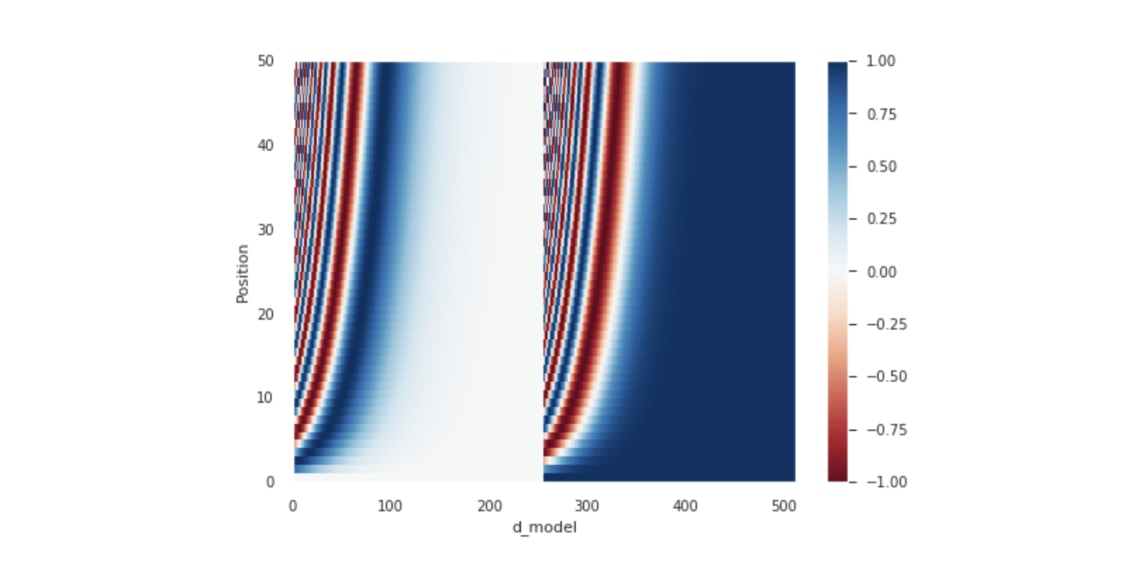### Encoder¶

Encoder 裡頭主要包含了 3 個元件：

• 輸入的詞嵌入層
• 位置編碼
• N 個 Encoder layers

class Encoder(tf.keras.layers.Layer):
# Encoder 的初始參數除了本來就要給 EncoderLayer 的參數還多了：
# - num_layers: 決定要有幾個 EncoderLayers, 前面影片中的 N
# - input_vocab_size: 用來把索引轉成詞嵌入向量
def __init__(self, num_layers, d_model, num_heads, dff, input_vocab_size,
rate=0.1):
super(Encoder, self).__init__()

self.d_model = d_model

self.embedding = tf.keras.layers.Embedding(input_vocab_size, d_model)
self.pos_encoding = positional_encoding(input_vocab_size, self.d_model)

# 建立 num_layers 個 EncoderLayers
self.enc_layers = [EncoderLayer(d_model, num_heads, dff, rate)
for _ in range(num_layers)]

self.dropout = tf.keras.layers.Dropout(rate)

def call(self, x, training, mask):
# 輸入的 x.shape == (batch_size, input_seq_len)
# 以下各 layer 的輸出皆為 (batch_size, input_seq_len, d_model)
input_seq_len = tf.shape(x)

# 將 2 維的索引序列轉成 3 維的詞嵌入張量，並依照論文乘上 sqrt(d_model)
# 再加上對應長度的位置編碼
x = self.embedding(x)
x *= tf.math.sqrt(tf.cast(self.d_model, tf.float32))
x += self.pos_encoding[:, :input_seq_len, :]

# 對 embedding 跟位置編碼的總合做 regularization
# 這在 Decoder 也會做
x = self.dropout(x, training=training)

# 通過 N 個 EncoderLayer 做編碼
for i, enc_layer in enumerate(self.enc_layers):
x = enc_layer(x, training, mask)
# 以下只是用來 demo EncoderLayer outputs
#print('-' * 20)
#print(f"EncoderLayer {i + 1}'s output:", x)

return x


# 超參數
num_layers = 2 # 2 層的 Encoder
d_model = 4
num_heads = 2
dff = 8
input_vocab_size = subword_encoder_en.vocab_size + 2 # 記得加上 <start>, <end>

# 初始化一個 Encoder
encoder = Encoder(num_layers, d_model, num_heads, dff, input_vocab_size)

# 將 2 維的索引序列丟入 Encoder 做編碼
enc_out = encoder(inp, training=False, mask=None)
print("inp:", inp)
print("-" * 20)
print("enc_out:", enc_out)

inp: tf.Tensor(
[[8135  105   10 1304 7925 8136    0    0]
[8135   17 3905 6013   12 2572 7925 8136]], shape=(2, 8), dtype=int64)
--------------------
enc_out: tf.Tensor(
[[[-0.80654097 -0.5846039  -0.31439844  1.7055433 ]
[-0.46891153 -0.57408124 -0.6840381   1.727031  ]
[-0.319709   -0.17782518 -1.1191479   1.616682  ]
[-0.49274105  0.26990706 -1.2412689   1.4641027 ]
[-0.88477194  0.16279429 -0.8493918   1.5713693 ]
[-0.96625364 -0.25279218 -0.4533522   1.6723981 ]
[-0.8476429  -0.5615218  -0.28872433  1.6978891 ]
[-0.61957765 -0.5919263  -0.51938564  1.7308894 ]]

[[-0.8083886  -0.56457365 -0.33460823  1.7075704 ]
[-0.50152016 -0.5214133  -0.7037289   1.7266623 ]
[-0.34244898 -0.11313835 -1.1444559   1.6000432 ]
[-0.5072439   0.21401608 -1.2050328   1.4982607 ]
[-0.88611245  0.26368466 -0.9036027   1.5260304 ]
[-0.96629447 -0.21083635 -0.49055386  1.6676848 ]
[-0.86832803 -0.5383212  -0.28836083  1.6950101 ]
[-0.6246328  -0.57586765 -0.5305909   1.7310913 ]]], shape=(2, 8, 4), dtype=float32)


• 輸入：（batch_size, seq_len）
• 輸出：（batch_size, seq_len, d_model）

### Decoder¶

Decoder layer 本來就只跟 Encoder layer 差在一個 MHA，而這邏輯被包起來以後呼叫它的 Decoder 做的事情就跟 Encoder 基本上沒有兩樣了。

class Decoder(tf.keras.layers.Layer):
# 初始參數跟 Encoder 只差在用 target_vocab_size 而非 inp_vocab_size
def __init__(self, num_layers, d_model, num_heads, dff, target_vocab_size,
rate=0.1):
super(Decoder, self).__init__()

self.d_model = d_model

# 為中文（目標語言）建立詞嵌入層
self.embedding = tf.keras.layers.Embedding(target_vocab_size, d_model)
self.pos_encoding = positional_encoding(target_vocab_size, self.d_model)

self.dec_layers = [DecoderLayer(d_model, num_heads, dff, rate)
for _ in range(num_layers)]
self.dropout = tf.keras.layers.Dropout(rate)

# 呼叫時的參數跟 DecoderLayer 一模一樣
def call(self, x, enc_output, training,
combined_mask, inp_padding_mask):

tar_seq_len = tf.shape(x)
attention_weights = {}  # 用來存放每個 Decoder layer 的注意權重

# 這邊跟 Encoder 做的事情完全一樣
x = self.embedding(x)  # (batch_size, tar_seq_len, d_model)
x *= tf.math.sqrt(tf.cast(self.d_model, tf.float32))
x += self.pos_encoding[:, :tar_seq_len, :]
x = self.dropout(x, training=training)

for i, dec_layer in enumerate(self.dec_layers):
x, block1, block2 = dec_layer(x, enc_output, training,
combined_mask, inp_padding_mask)

# 將從每個 Decoder layer 取得的注意權重全部存下來回傳，方便我們觀察
attention_weights['decoder_layer{}_block1'.format(i + 1)] = block1
attention_weights['decoder_layer{}_block2'.format(i + 1)] = block2

# x.shape == (batch_size, tar_seq_len, d_model)
return x, attention_weights


# 超參數
num_layers = 2 # 2 層的 Decoder
d_model = 4
num_heads = 2
dff = 8
target_vocab_size = subword_encoder_zh.vocab_size + 2 # 記得加上 <start>, <end>

# 遮罩
inp_padding_mask = create_padding_mask(inp)
tar_padding_mask = create_padding_mask(tar)
look_ahead_mask = create_look_ahead_mask(tar.shape)
combined_mask = tf.math.maximum(tar_padding_mask, look_ahead_mask)

# 初始化一個 Decoder
decoder = Decoder(num_layers, d_model, num_heads, dff, target_vocab_size)

# 將 2 維的索引序列以及遮罩丟入 Decoder
print("tar:", tar)
print("-" * 20)
print("combined_mask:", combined_mask)
print("-" * 20)
print("enc_out:", enc_out)
print("-" * 20)
print("inp_padding_mask:", inp_padding_mask)
print("-" * 20)
dec_out, attn = decoder(tar, enc_out, training=False,
combined_mask=combined_mask,
inp_padding_mask=inp_padding_mask)
print("dec_out:", dec_out)
print("-" * 20)
for block_name, attn_weights in attn.items():
print(f"{block_name}.shape: {attn_weights.shape}")

tar: tf.Tensor(
[[4201   10  241   80   27    3 4202    0    0    0]
[4201  162  467  421  189   14    7  553    3 4202]], shape=(2, 10), dtype=int64)
--------------------
combined_mask: tf.Tensor(
[[[[0. 1. 1. 1. 1. 1. 1. 1. 1. 1.]
[0. 0. 1. 1. 1. 1. 1. 1. 1. 1.]
[0. 0. 0. 1. 1. 1. 1. 1. 1. 1.]
[0. 0. 0. 0. 1. 1. 1. 1. 1. 1.]
[0. 0. 0. 0. 0. 1. 1. 1. 1. 1.]
[0. 0. 0. 0. 0. 0. 1. 1. 1. 1.]
[0. 0. 0. 0. 0. 0. 0. 1. 1. 1.]
[0. 0. 0. 0. 0. 0. 0. 1. 1. 1.]
[0. 0. 0. 0. 0. 0. 0. 1. 1. 1.]
[0. 0. 0. 0. 0. 0. 0. 1. 1. 1.]]]

[[[0. 1. 1. 1. 1. 1. 1. 1. 1. 1.]
[0. 0. 1. 1. 1. 1. 1. 1. 1. 1.]
[0. 0. 0. 1. 1. 1. 1. 1. 1. 1.]
[0. 0. 0. 0. 1. 1. 1. 1. 1. 1.]
[0. 0. 0. 0. 0. 1. 1. 1. 1. 1.]
[0. 0. 0. 0. 0. 0. 1. 1. 1. 1.]
[0. 0. 0. 0. 0. 0. 0. 1. 1. 1.]
[0. 0. 0. 0. 0. 0. 0. 0. 1. 1.]
[0. 0. 0. 0. 0. 0. 0. 0. 0. 1.]
[0. 0. 0. 0. 0. 0. 0. 0. 0. 0.]]]], shape=(2, 1, 10, 10), dtype=float32)
--------------------
enc_out: tf.Tensor(
[[[-0.80654097 -0.5846039  -0.31439844  1.7055433 ]
[-0.46891153 -0.57408124 -0.6840381   1.727031  ]
[-0.319709   -0.17782518 -1.1191479   1.616682  ]
[-0.49274105  0.26990706 -1.2412689   1.4641027 ]
[-0.88477194  0.16279429 -0.8493918   1.5713693 ]
[-0.96625364 -0.25279218 -0.4533522   1.6723981 ]
[-0.8476429  -0.5615218  -0.28872433  1.6978891 ]
[-0.61957765 -0.5919263  -0.51938564  1.7308894 ]]

[[-0.8083886  -0.56457365 -0.33460823  1.7075704 ]
[-0.50152016 -0.5214133  -0.7037289   1.7266623 ]
[-0.34244898 -0.11313835 -1.1444559   1.6000432 ]
[-0.5072439   0.21401608 -1.2050328   1.4982607 ]
[-0.88611245  0.26368466 -0.9036027   1.5260304 ]
[-0.96629447 -0.21083635 -0.49055386  1.6676848 ]
[-0.86832803 -0.5383212  -0.28836083  1.6950101 ]
[-0.6246328  -0.57586765 -0.5305909   1.7310913 ]]], shape=(2, 8, 4), dtype=float32)
--------------------
inp_padding_mask: tf.Tensor(
[[[[0. 0. 0. 0. 0. 0. 1. 1.]]]

[[[0. 0. 0. 0. 0. 0. 0. 0.]]]], shape=(2, 1, 1, 8), dtype=float32)
--------------------
dec_out: tf.Tensor(
[[[-0.5437632  -1.055963    1.6090912  -0.0093651 ]
[-0.35729456 -1.2363737   1.5295789   0.06408926]
[ 0.35950443 -1.4217519   1.3327445  -0.27049693]
[ 0.00910451 -1.3681054   1.4556323  -0.09663116]
[-0.39842203 -1.0891637   1.6237149  -0.13612938]
[-0.41910946 -1.0254465   1.6521797  -0.20762381]
[-0.36797434 -1.036104    1.6521349  -0.2480565 ]
[-0.19375193 -1.1218892   1.6165614  -0.30092025]
[ 0.40127647 -1.3597702   1.3540744  -0.39558053]
[ 0.17590097 -1.419068    1.3905344  -0.14736754]]

[[-0.54991776 -1.0509207   1.6102997  -0.00946123]
[-0.3790077  -1.2450974   1.514628    0.10947719]
[ 0.1746773  -1.3877552   1.415193   -0.20211506]
[-0.03870562 -1.3375971   1.4825788  -0.10627584]
[-0.43508232 -1.067575    1.6293938  -0.12673649]
[-0.41048303 -1.0317237   1.6503688  -0.20816201]
[-0.3626595  -1.0360833   1.652463   -0.25372016]
[-0.24817836 -1.1092765   1.6238651  -0.26641032]
[ 0.1850568  -1.3670969   1.4271388  -0.2450987 ]
[ 0.09142628 -1.3988855   1.4218552  -0.11439597]]], shape=(2, 10, 4), dtype=float32)
--------------------
decoder_layer1_block1.shape: (2, 2, 10, 10)
decoder_layer1_block2.shape: (2, 2, 10, 8)
decoder_layer2_block1.shape: (2, 2, 10, 10)
decoder_layer2_block2.shape: (2, 2, 10, 8)


• 初始時給它中文（目標語言）的字典大小、其他超參數
• 輸入中文 batch 的索引序列
• 也要輸入兩個遮罩以及 Encoder 輸出 enc_out

Decoder 的輸出你現在應該都可以很輕鬆地解讀才是。基本上跟 Decoder layer 一模一樣，只差在我們額外輸出一個 Python dict，裡頭存放所有 Decoder layers 的注意權重。

### 第一個 Transformer¶

Transformer 本身只有 3 個 layers

1. Encoder
2. Decoder
3. Final linear layer

# Transformer 之上已經沒有其他 layers 了，我們使用 tf.keras.Model 建立一個模型
class Transformer(tf.keras.Model):
# 初始參數包含 Encoder & Decoder 都需要超參數以及中英字典數目
def __init__(self, num_layers, d_model, num_heads, dff, input_vocab_size,
target_vocab_size, rate=0.1):
super(Transformer, self).__init__()

self.encoder = Encoder(num_layers, d_model, num_heads, dff,
input_vocab_size, rate)

self.decoder = Decoder(num_layers, d_model, num_heads, dff,
target_vocab_size, rate)
# 這個 FFN 輸出跟中文字典一樣大的 logits 數，等通過 softmax 就代表每個中文字的出現機率
self.final_layer = tf.keras.layers.Dense(target_vocab_size)

# enc_padding_mask 跟 dec_padding_mask 都是英文序列的 padding mask，
# 只是一個給 Encoder layer 的 MHA 用，一個是給 Decoder layer 的 MHA 2 使用
def call(self, inp, tar, training, enc_padding_mask,
combined_mask, dec_padding_mask):

enc_output = self.encoder(inp, training, enc_padding_mask)  # (batch_size, inp_seq_len, d_model)

# dec_output.shape == (batch_size, tar_seq_len, d_model)
dec_output, attention_weights = self.decoder(
tar, enc_output, training, combined_mask, dec_padding_mask)

# 將 Decoder 輸出通過最後一個 linear layer
final_output = self.final_layer(dec_output)  # (batch_size, tar_seq_len, target_vocab_size)

return final_output, attention_weights


• 輸入：
• 英文序列：（batch_size, inp_seq_len）
• 中文序列：（batch_size, tar_seq_len）
• 輸出：
• 生成序列：（batch_size, tar_seq_len, target_vocab_size）
• 注意權重的 dict

# 超參數
num_layers = 1
d_model = 4
num_heads = 2
dff = 8

# + 2 是為了 <start> & <end> token
input_vocab_size = subword_encoder_en.vocab_size + 2
output_vocab_size = subword_encoder_zh.vocab_size + 2

# 重點中的重點。訓練時用前一個字來預測下一個中文字
tar_inp = tar[:, :-1]
tar_real = tar[:, 1:]

# 來源 / 目標語言用的遮罩。注意 comined_mask 已經將目標語言的兩種遮罩合而為一
inp_padding_mask = create_padding_mask(inp)
tar_padding_mask = create_padding_mask(tar_inp)
look_ahead_mask = create_look_ahead_mask(tar_inp.shape)
combined_mask = tf.math.maximum(tar_padding_mask, look_ahead_mask)

# 初始化我們的第一個 transformer
transformer = Transformer(num_layers, d_model, num_heads, dff,
input_vocab_size, output_vocab_size)

# 將英文、中文序列丟入取得 Transformer 預測下個中文字的結果
predictions, attn_weights = transformer(inp, tar_inp, False, inp_padding_mask,
combined_mask, inp_padding_mask)

print("tar:", tar)
print("-" * 20)
print("tar_inp:", tar_inp)
print("-" * 20)
print("tar_real:", tar_real)
print("-" * 20)
print("predictions:", predictions)

tar: tf.Tensor(
[[4201   10  241   80   27    3 4202    0    0    0]
[4201  162  467  421  189   14    7  553    3 4202]], shape=(2, 10), dtype=int64)
--------------------
tar_inp: tf.Tensor(
[[4201   10  241   80   27    3 4202    0    0]
[4201  162  467  421  189   14    7  553    3]], shape=(2, 9), dtype=int64)
--------------------
tar_real: tf.Tensor(
[[  10  241   80   27    3 4202    0    0    0]
[ 162  467  421  189   14    7  553    3 4202]], shape=(2, 9), dtype=int64)
--------------------
predictions: tf.Tensor(
[[[ 0.00929452 -0.01123782  0.05421777 ... -0.01170466  0.00628542
-0.07576236]
[ 0.03640017 -0.01885041  0.05113849 ... -0.02349908  0.01716622
-0.06729948]
[ 0.05617092 -0.02265774  0.04667147 ... -0.02913139  0.0241506
-0.05331099]
...
[ 0.00905135 -0.01058669  0.05486142 ... -0.01039154  0.0058039
-0.07445519]
[ 0.02215609 -0.01478041  0.05375389 ... -0.0170105   0.01135763
-0.07241639]
[ 0.0478656  -0.02148081  0.04837158 ... -0.02759764  0.02148173
-0.06043392]]

[[ 0.00996658 -0.01115559  0.05453676 ... -0.0114185   0.00637141
-0.07500792]
[ 0.03897631 -0.01930442  0.0508956  ... -0.02409907  0.01803425
-0.0656432 ]
[ 0.05387272 -0.02244362  0.04702405 ... -0.02893805  0.02348556
-0.05554678]
...
[ 0.01048942 -0.01085559  0.05502523 ... -0.01070841  0.0062833
-0.07385261]
[ 0.02370835 -0.01504852  0.05381611 ... -0.01732858  0.01186723
-0.07158875]
[ 0.04920105 -0.02166032  0.0481827  ... -0.02781233  0.02190085
-0.05933255]]], shape=(2, 9, 4203), dtype=float32)


...

tar_inp = tar[:, :-1]
tar_real = tar[:, 1:]

predictions, attn_weights = transformer(inp, tar_inp, False, ...)

...


## 定義損失函數與指標¶

loss_object = tf.keras.losses.SparseCategoricalCrossentropy(
from_logits=True, reduction='none')

# 假設我們要解的是一個 binary classifcation， 0 跟 1 個代表一個 label
real = tf.constant([1, 1, 0], shape=(1, 3), dtype=tf.float32)
pred = tf.constant([[0, 1], [0, 1], [0, 1]], dtype=tf.float32)
loss_object(real, pred)

<tf.Tensor: id=197487, shape=(3,), dtype=float32, numpy=array([0.31326166, 0.31326166, 1.3132616 ], dtype=float32)>

• real：一個包含 N 個正確 labels 的序列
• pred：一個包含 N 個維度為 label 數的 logit 序列

print("predictions:", predictions)
print("-" * 20)
print(tf.reduce_sum(predictions, axis=-1))

predictions: tf.Tensor(
[[[ 0.00929452 -0.01123782  0.05421777 ... -0.01170466  0.00628542
-0.07576236]
[ 0.03640017 -0.01885041  0.05113849 ... -0.02349908  0.01716622
-0.06729948]
[ 0.05617092 -0.02265774  0.04667147 ... -0.02913139  0.0241506
-0.05331099]
...
[ 0.00905135 -0.01058669  0.05486142 ... -0.01039154  0.0058039
-0.07445519]
[ 0.02215609 -0.01478041  0.05375389 ... -0.0170105   0.01135763
-0.07241639]
[ 0.0478656  -0.02148081  0.04837158 ... -0.02759764  0.02148173
-0.06043392]]

[[ 0.00996658 -0.01115559  0.05453676 ... -0.0114185   0.00637141
-0.07500792]
[ 0.03897631 -0.01930442  0.0508956  ... -0.02409907  0.01803425
-0.0656432 ]
[ 0.05387272 -0.02244362  0.04702405 ... -0.02893805  0.02348556
-0.05554678]
...
[ 0.01048942 -0.01085559  0.05502523 ... -0.01070841  0.0062833
-0.07385261]
[ 0.02370835 -0.01504852  0.05381611 ... -0.01732858  0.01186723
-0.07158875]
[ 0.04920105 -0.02166032  0.0481827  ... -0.02781233  0.02190085
-0.05933255]]], shape=(2, 9, 4203), dtype=float32)
--------------------
tf.Tensor(
[[1.4971986 3.1899047 4.1454954 3.7353938 2.869739  1.8605256 1.3746347
2.2779167 3.8190796]
[1.4881071 3.303587  4.0757227 3.7524652 2.836317  1.9132937 1.4376438
2.3432927 3.8689976]], shape=(2, 9), dtype=float32)


def loss_function(real, pred):
# 這次的 mask 將序列中不等於 0 的位置視為 1，其餘為 0
mask = tf.math.logical_not(tf.math.equal(real, 0))
# 照樣計算所有位置的 cross entropy 但不加總
loss_ = loss_object(real, pred)
mask = tf.cast(mask, dtype=loss_.dtype)
loss_ *= mask  # 只計算非 <pad> 位置的損失

return tf.reduce_mean(loss_)


train_loss = tf.keras.metrics.Mean(name='train_loss')
train_accuracy = tf.keras.metrics.SparseCategoricalAccuracy(
name='train_accuracy')


## 設置超參數¶

• num_layers 決定 Transfomer 裡頭要有幾個 Encoder / Decoder layers
• d_model 決定我們子詞的 representation space 維度
• num_heads 要做幾頭的自注意力運算
• dff 決定 FFN 的中間維度
• dropout_rate 預設 0.1，一般用預設值即可
• input_vocab_size：輸入語言（英文）的字典大小
• target_vocab_size：輸出語言（中文）的字典大小

• num_layers=6
• d_model=512
• dff=2048

num_layers = 4
d_model = 128
dff = 512
num_heads = 8

input_vocab_size = subword_encoder_en.vocab_size + 2
target_vocab_size = subword_encoder_zh.vocab_size + 2
dropout_rate = 0.1  # 預設值

print("input_vocab_size:", input_vocab_size)
print("target_vocab_size:", target_vocab_size)

input_vocab_size: 8137
target_vocab_size: 4203


4 層 Encoder / Decoder layers 不算貪心，小巫見大巫（笑

## 設置 Optimizer¶class CustomSchedule(tf.keras.optimizers.schedules.LearningRateSchedule):
# 論文預設 warmup_steps = 4000
def __init__(self, d_model, warmup_steps=4000):
super(CustomSchedule, self).__init__()

self.d_model = d_model
self.d_model = tf.cast(self.d_model, tf.float32)

self.warmup_steps = warmup_steps

def __call__(self, step):
arg1 = tf.math.rsqrt(step)
arg2 = step * (self.warmup_steps ** -1.5)

return tf.math.rsqrt(self.d_model) * tf.math.minimum(arg1, arg2)

# 將客製化 learning rate schdeule 丟入 Adam opt.
# Adam opt. 的參數都跟論文相同
learning_rate = CustomSchedule(d_model)
optimizer = tf.keras.optimizers.Adam(learning_rate, beta_1=0.9, beta_2=0.98,
epsilon=1e-9)


d_models = [128, 256, 512]
warmup_steps = [1000 * i for i in range(1, 4)]

schedules = []
labels = []
colors = ["blue", "red", "black"]
for d in d_models:
schedules += [CustomSchedule(d, s) for s in warmup_steps]
labels += [f"d_model: {d}, warm: {s}" for s in warmup_steps]

for i, (schedule, label) in enumerate(zip(schedules, labels)):
plt.plot(schedule(tf.range(10000, dtype=tf.float32)),
label=label, color=colors[i // 3])

plt.legend()

plt.ylabel("Learning Rate")
plt.xlabel("Train Step")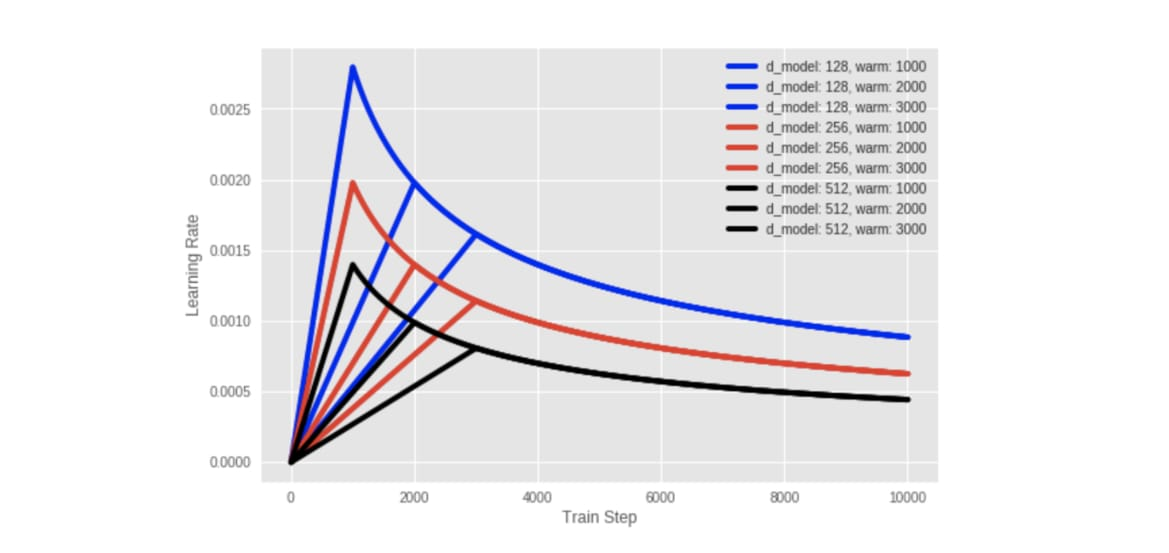## 實際訓練以及定時存檔¶

transformer = Transformer(num_layers, d_model, num_heads, dff,
input_vocab_size, target_vocab_size, dropout_rate)

print(f"""這個 Transformer 有 {num_layers} 層 Encoder / Decoder layers
d_model: {d_model}
num_heads: {num_heads}
dff: {dff}
input_vocab_size: {input_vocab_size}
target_vocab_size: {target_vocab_size}
dropout_rate: {dropout_rate}

""")

這個 Transformer 有 4 層 Encoder / Decoder layers
d_model: 128
num_heads: 8
dff: 512
input_vocab_size: 8137
target_vocab_size: 4203
dropout_rate: 0.1



# 方便比較不同實驗/ 不同超參數設定的結果
run_id = f"{num_layers}layers_{d_model}d_{num_heads}heads_{dff}dff_{train_perc}train_perc"
checkpoint_path = os.path.join(checkpoint_path, run_id)
log_dir = os.path.join(log_dir, run_id)

# tf.train.Checkpoint 可以幫我們把想要存下來的東西整合起來，方便儲存與讀取
# 一般來說你會想存下模型以及 optimizer 的狀態
ckpt = tf.train.Checkpoint(transformer=transformer,
optimizer=optimizer)

# ckpt_manager 會去 checkpoint_path 看有沒有符合 ckpt 裡頭定義的東西
# 存檔的時候只保留最近 5 次 checkpoints，其他自動刪除
ckpt_manager = tf.train.CheckpointManager(ckpt, checkpoint_path, max_to_keep=5)

# 如果在 checkpoint 路徑上有發現檔案就讀進來
if ckpt_manager.latest_checkpoint:
ckpt.restore(ckpt_manager.latest_checkpoint)

# 用來確認之前訓練多少 epochs 了
last_epoch = int(ckpt_manager.latest_checkpoint.split("-")[-1])
print(f'已讀取最新的 checkpoint，模型已訓練 {last_epoch} epochs。')
else:
last_epoch = 0
print("沒找到 checkpoint，從頭訓練。")

已讀取最新的 checkpoint，模型已訓練 50 epochs。「誒！？ 你不當場訓練嗎？」「直接載入已訓練的模型太狗了吧！」

# 為 Transformer 的 Encoder / Decoder 準備遮罩
def create_masks(inp, tar):
# 英文句子的 padding mask，要交給 Encoder layer 自注意力機制用的
enc_padding_mask = create_padding_mask(inp)

# 同樣也是英文句子的 padding mask，但是是要交給 Decoder layer 的 MHA 2
# 關注 Encoder 輸出序列用的
dec_padding_mask = create_padding_mask(inp)

# Decoder layer 的 MHA1 在做自注意力機制用的
# combined_mask 是中文句子的 padding mask 跟 look ahead mask 的疊加
look_ahead_mask = create_look_ahead_mask(tf.shape(tar))
dec_target_padding_mask = create_padding_mask(tar)
combined_mask = tf.maximum(dec_target_padding_mask, look_ahead_mask)

return enc_padding_mask, combined_mask, dec_padding_mask


1. 對訓練數據做些必要的前處理
2. 將數據丟入模型，取得預測結果
3. 用預測結果跟正確解答計算 loss
4. 取出梯度並利用 optimizer 做梯度下降

@tf.function  # 讓 TensorFlow 幫我們將 eager code 優化並加快運算
def train_step(inp, tar):
# 前面說過的，用去尾的原始序列去預測下一個字的序列
tar_inp = tar[:, :-1]
tar_real = tar[:, 1:]

# 建立 3 個遮罩
enc_padding_mask, combined_mask, dec_padding_mask = create_masks(inp, tar_inp)

# 紀錄 Transformer 的所有運算過程以方便之後做梯度下降
with tf.GradientTape() as tape:
# 注意是丟入 tar_inp 而非 tar。記得將 training 參數設定為 True
predictions, _ = transformer(inp, tar_inp,
True,
enc_padding_mask,
combined_mask,
dec_padding_mask)
# 跟影片中顯示的相同，計算左移一個字的序列跟模型預測分佈之間的差異，當作 loss
loss = loss_function(tar_real, predictions)

# 取出梯度並呼叫前面定義的 Adam optimizer 幫我們更新 Transformer 裡頭可訓練的參數
gradients = tape.gradient(loss, transformer.trainable_variables)
optimizer.apply_gradients(zip(gradients, transformer.trainable_variables))

# 將 loss 以及訓練 acc 記錄到 TensorBoard 上，非必要
train_loss(loss)
train_accuracy(tar_real, predictions)


• 對輸入數據做些前處理（本文中的遮罩、將輸出序列左移當成正解 etc.）
• 利用 tf.GradientTape 輕鬆記錄數據被模型做的所有轉換並計算 loss
• 將梯度取出並讓 optimzier 對可被訓練的權重做梯度下降（上升）

# 定義我們要看幾遍數據集
EPOCHS = 30
print(f"此超參數組合的 Transformer 已經訓練 {last_epoch} epochs。")
print(f"剩餘 epochs：{min(0, last_epoch - EPOCHS)}")

# 用來寫資訊到 TensorBoard，非必要但十分推薦
summary_writer = tf.summary.create_file_writer(log_dir)

# 比對設定的 EPOCHS 以及已訓練的 last_epoch 來決定還要訓練多少 epochs
for epoch in range(last_epoch, EPOCHS):
start = time.time()

# 重置紀錄 TensorBoard 的 metrics
train_loss.reset_states()
train_accuracy.reset_states()

# 一個 epoch 就是把我們定義的訓練資料集一個一個 batch 拿出來處理，直到看完整個數據集
for (step_idx, (inp, tar)) in enumerate(train_dataset):

# 每次 step 就是將數據丟入 Transformer，讓它生預測結果並計算梯度最小化 loss
train_step(inp, tar)

# 每個 epoch 完成就存一次檔
if (epoch + 1) % 1 == 0:
ckpt_save_path = ckpt_manager.save()
print ('Saving checkpoint for epoch {} at {}'.format(epoch+1,
ckpt_save_path))

# 將 loss 以及 accuracy 寫到 TensorBoard 上
with summary_writer.as_default():
tf.summary.scalar("train_loss", train_loss.result(), step=epoch + 1)
tf.summary.scalar("train_acc", train_accuracy.result(), step=epoch + 1)

print('Epoch {} Loss {:.4f} Accuracy {:.4f}'.format(epoch + 1,
train_loss.result(),
train_accuracy.result()))
print('Time taken for 1 epoch: {} secs\n'.format(time.time() - start))

此超參數組合的 Transformer 已經訓練 50 epochs。



• （非必要）重置寫到 TensorBoard 的 metrics 的值
• 將整個數據集的 batch 取出，交給 train_step 函式處理
• （非必要）存 checkpoints
• （非必要）將當前 epoch 結果寫到 TensorBoard
• （非必要）在標準輸出顯示當前 epoch 結果

# 87 分，不能再高了。
for epoch in range(EPOCHS):
for inp, tar in train_dataset:
train_step(inp, tar)Saving checkpoint for epoch 1 at nmt/checkpoints/4layers_128d_8heads_512dff_20train_perc/ckpt-1
Epoch 1 Loss 5.2072 Accuracy 0.0179
Time taken for 1 epoch: 206.54558181762695 secs

Saving checkpoint for epoch 2 at nmt/checkpoints/4layers_128d_8heads_512dff_20train_perc/ckpt-2
Epoch 2 Loss 4.2652 Accuracy 0.0560
Time taken for 1 epoch: 68.48831677436829 secs

Saving checkpoint for epoch 3 at nmt/checkpoints/4layers_128d_8heads_512dff_20train_perc/ckpt-3
Epoch 3 Loss 3.7987 Accuracy 0.0910
Time taken for 1 epoch: 68.41022562980652 secs

...

Saving checkpoint for epoch 29 at nmt/checkpoints/4layers_128d_8heads_512dff_20train_perc/ckpt-29
Epoch 29 Loss 1.2693 Accuracy 0.3929
Time taken for 1 epoch: 69.18679404258728 secs

Saving checkpoint for epoch 30 at nmt/checkpoints/4layers_128d_8heads_512dff_20train_perc/ckpt-30
Epoch 30 Loss 1.2426 Accuracy 0.3965
Time taken for 1 epoch: 68.7313539981842 secs



%load_ext tensorboard
%tensorboard --logdir {your_log_dir}


## 實際進行英翻中¶

• 將輸入的英文句子利用 Subword Tokenizer 轉換成子詞索引序列（還記得 inp 吧？）
• 在該英文索引序列前後加上代表英文 BOS / EOS 的 tokens
• 在 Transformer 輸出序列長度達到 MAX_LENGTH 之前重複以下步驟：
• 為目前已經生成的中文索引序列產生新的遮罩
• 將剛剛的英文序列、當前的中文序列以及各種遮罩放入 Transformer
• 將 Transformer 輸出序列的最後一個位置的向量取出，並取 argmax 取得新的預測中文索引
• 將此索引加到目前的中文索引序列裡頭作為 Transformer 到此為止的輸出結果
• 如果新生成的中文索引為 <end> 則代表中文翻譯已全部生成完畢，直接回傳
• 將最後得到的中文索引序列回傳作為翻譯結果

# 給定一個英文句子，輸出預測的中文索引數字序列以及注意權重 dict
def evaluate(inp_sentence):

# 準備英文句子前後會加上的 <start>, <end>
start_token = [subword_encoder_en.vocab_size]
end_token = [subword_encoder_en.vocab_size + 1]

# inp_sentence 是字串，我們用 Subword Tokenizer 將其變成子詞的索引序列
# 並在前後加上 BOS / EOS
inp_sentence = start_token + subword_encoder_en.encode(inp_sentence) + end_token
encoder_input = tf.expand_dims(inp_sentence, 0)

# 跟我們在影片裡看到的一樣，Decoder 在第一個時間點吃進去的輸入
# 是一個只包含一個中文 <start> token 的序列
decoder_input = [subword_encoder_zh.vocab_size]
output = tf.expand_dims(decoder_input, 0)  # 增加 batch 維度

# auto-regressive，一次生成一個中文字並將預測加到輸入再度餵進 Transformer
for i in range(MAX_LENGTH):
# 每多一個生成的字就得產生新的遮罩
enc_padding_mask, combined_mask, dec_padding_mask = create_masks(
encoder_input, output)

# predictions.shape == (batch_size, seq_len, vocab_size)
predictions, attention_weights = transformer(encoder_input,
output,
False,
enc_padding_mask,
combined_mask,
dec_padding_mask)

# 將序列中最後一個 distribution 取出，並將裡頭值最大的當作模型最新的預測字
predictions = predictions[: , -1:, :]  # (batch_size, 1, vocab_size)

predicted_id = tf.cast(tf.argmax(predictions, axis=-1), tf.int32)

# 遇到 <end> token 就停止回傳，代表模型已經產生完結果
if tf.equal(predicted_id, subword_encoder_zh.vocab_size + 1):
return tf.squeeze(output, axis=0), attention_weights

#將 Transformer 新預測的中文索引加到輸出序列中，讓 Decoder 可以在產生
# 下個中文字的時候關注到最新的 predicted_id
output = tf.concat([output, predicted_id], axis=-1)

# 將 batch 的維度去掉後回傳預測的中文索引序列
return tf.squeeze(output, axis=0), attention_weights


# 要被翻譯的英文句子
sentence = "China, India, and others have enjoyed continuing economic growth."

# 取得預測的中文索引序列
predicted_seq, _ = evaluate(sentence)

# 過濾掉 <start> & <end> tokens 並用中文的 subword tokenizer 幫我們將索引序列還原回中文句子
target_vocab_size = subword_encoder_zh.vocab_size
predicted_seq_without_bos_eos = [idx for idx in predicted_seq if idx < target_vocab_size]
predicted_sentence = subword_encoder_zh.decode(predicted_seq_without_bos_eos)

print("sentence:", sentence)
print("-" * 20)
print("predicted_seq:", predicted_seq)
print("-" * 20)
print("predicted_sentence:", predicted_sentence)

sentence: China, India, and others have enjoyed continuing economic growth.
--------------------
predicted_seq: tf.Tensor(
[4201   16    4   37  386  101    8   34   32    4   33  110  956  186
14   22   52  107   84    1  104  292   49  218    3], shape=(25,), dtype=int32)
--------------------
predicted_sentence: 中国、印度和其他国家都享受了经济增长的持续发展。


transformer.summary()

Model: "transformer_1"
_________________________________________________________________
Layer (type)                 Output Shape              Param #
=================================================================
encoder_2 (Encoder)          multiple                  1834624
_________________________________________________________________
decoder_2 (Decoder)          multiple                  1596288
_________________________________________________________________
dense_137 (Dense)            multiple                  542187
=================================================================
Total params: 3,973,099
Trainable params: 3,973,099
Non-trainable params: 0
_________________________________________________________________


## 視覺化注意權重¶

predicted_seq, attention_weights = evaluate(sentence)

# 在這邊我們自動選擇最後一個 Decoder layer 的 MHA 2，也就是 Decoder 關注 Encoder 的 MHA
layer_name = f"decoder_layer{num_layers}_block2"

print("sentence:", sentence)
print("-" * 20)
print("predicted_seq:", predicted_seq)
print("-" * 20)
print("attention_weights.keys():")
for layer_name, attn in attention_weights.items():
print(f"{layer_name}.shape: {attn.shape}")
print("-" * 20)
print("layer_name:", layer_name)

sentence: China, India, and others have enjoyed continuing economic growth.
--------------------
predicted_seq: tf.Tensor(
[4201   16    4   37  386  101    8   34   32    4   33  110  956  186
14   22   52  107   84    1  104  292   49  218    3], shape=(25,), dtype=int32)
--------------------
attention_weights.keys():
decoder_layer1_block1.shape: (1, 8, 25, 25)
decoder_layer1_block2.shape: (1, 8, 25, 15)
decoder_layer2_block1.shape: (1, 8, 25, 25)
decoder_layer2_block2.shape: (1, 8, 25, 15)
decoder_layer3_block1.shape: (1, 8, 25, 25)
decoder_layer3_block2.shape: (1, 8, 25, 15)
decoder_layer4_block1.shape: (1, 8, 25, 25)
decoder_layer4_block2.shape: (1, 8, 25, 15)
--------------------
layer_name: decoder_layer4_block2


block1 代表是 Decoder layer 自己關注自己的 MHA 1，因此倒數兩個維度都跟中文序列長度相同；block2 則是 Decoder layer 用來關注 Encoder 輸出的 MHA 2 ，在這邊我們選擇最後一個 Decoder layer 的 MHA 2 來看 Transformer 在生成中文序列時關注在英文句子的那些位置。

import matplotlib as mpl
# 你可能會需要自行下載一個中文字體檔案以讓 matplotlib 正確顯示中文
zhfont = mpl.font_manager.FontProperties(fname='/usr/share/fonts/SimHei/simhei.ttf')
plt.style.use("seaborn-whitegrid")

# 這個函式將英 -> 中翻譯的注意權重視覺化（注意：我們將注意權重 transpose 以最佳化渲染結果
def plot_attention_weights(attention_weights, sentence, predicted_seq, layer_name, max_len_tar=None):

fig = plt.figure(figsize=(17, 7))

sentence = subword_encoder_en.encode(sentence)

# 只顯示中文序列前 max_len_tar 個字以避免畫面太過壅擠
if max_len_tar:
predicted_seq = predicted_seq[:max_len_tar]
else:
max_len_tar = len(predicted_seq)

# 將某一個特定 Decoder layer 裡頭的 MHA 1 或 MHA2 的注意權重拿出來並去掉 batch 維度
attention_weights = tf.squeeze(attention_weights[layer_name], axis=0)
# (num_heads, tar_seq_len, inp_seq_len)

# 將每個 head 的注意權重畫出
for head in range(attention_weights.shape):
ax = fig.add_subplot(2, 4, head + 1)

# [注意]我為了將長度不短的英文子詞顯示在 y 軸，將注意權重做了 transpose
attn_map = np.transpose(attention_weights[head][:max_len_tar, :])
ax.matshow(attn_map, cmap='viridis')  # (inp_seq_len, tar_seq_len)

fontdict = {"fontproperties": zhfont}

ax.set_xticks(range(max(max_len_tar, len(predicted_seq))))
ax.set_xlim(-0.5, max_len_tar -1.5)

ax.set_yticks(range(len(sentence) + 2))
ax.set_xticklabels([subword_encoder_zh.decode([i]) for i in predicted_seq
if i < subword_encoder_zh.vocab_size],
fontdict=fontdict, fontsize=18)

ax.set_yticklabels(
['<start>'] + [subword_encoder_en.decode([i]) for i in sentence] + ['<end>'],
fontdict=fontdict)

ax.set_xlabel('Head {}'.format(head + 1))
ax.tick_params(axis="x", labelsize=12)
ax.tick_params(axis="y", labelsize=12)

plt.tight_layout()
plt.show()
plt.close(fig)


(batch_size, num_heads, zh_seq_len, en_seq_len)


plot_attention_weights(attention_weights, sentence,
predicted_seq, layer_name, max_len_tar=18)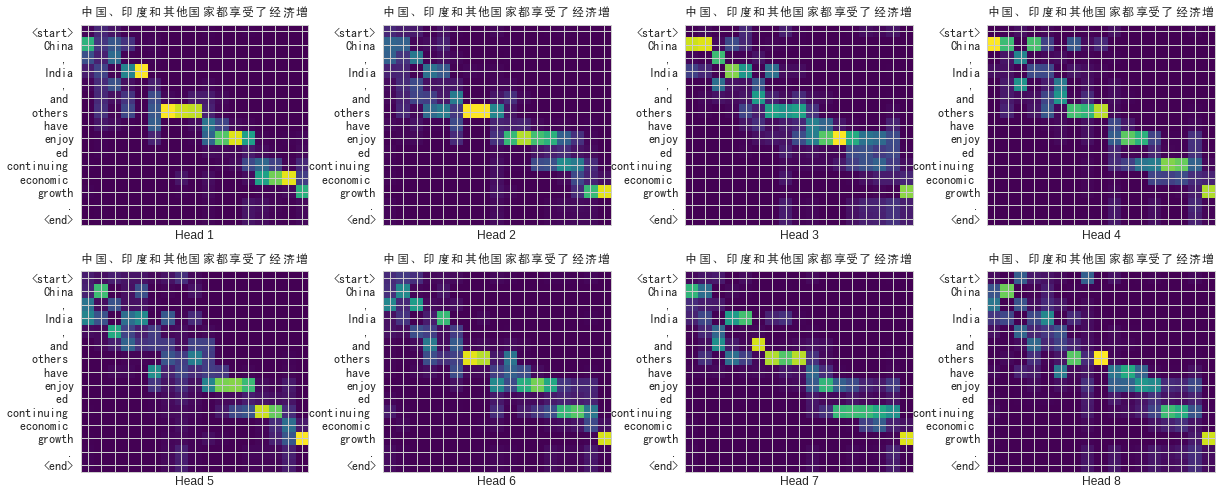Transformer 在訓練過程中逐漸學會關注在對的位置

## 在你離開之前¶